Amin
Topic Author
Posts: 2416
Joined: July 14th, 2002, 3:00 am

### Re: Breakthrough in the theory of stochastic differential equations and their simulation

Friends, in the program I posted and in my previous description, I have used our bivariate random variable V(Z_x,Z_y) as a product of X and Y (asset and volatility).
But since only the asset itself has bivariate dependence on both Z_x and Z_y, we could have also used only asset as a function of Z_x and Z_y or X(Z_x,Z_y) in the inner product calculations of coefficients of 2D hermites.
I will urge friends to try only SV asset X(k,l) on the 2D grid in the inner product with 2D hermites as opposed to product of X and Y. I will try to come up with a program on it soon.
Especially in SV equations, the equation of asset has dependence on both stochastic volatility and previous time value of the asset itself and asset has bivariate dependence (on Z_x and Z_y)  but asset SDE is certainly not a product of asset and stochastic volatility. Even though,if we want, we can easily construct a bivariate density of the product of asset and volatility by combining the SDEs of asset and volatility and their Z-series.
So in my previous program the bivariate random variable was product of asset and volatility but it could just have been bivariate value of only the asset. At that time, I did not have insight that I have slowly gained later so as to model the bivariate density of only asset as a function of Z_x and Z_y. I will try modifying my program for only bivariate representation of asset X and try posting it in a day or two.
So I suppose that every random variable that has bivariate dependence that is possibly product of two dependent random variables or just a single random variable that has bivariate dependence, both possiblities can be represented as a 2D hermite series. Our derivation of 2D hermite series coefficients only requires a bivariate dependence of the random variable.
You think life is a secret, Life is only love of flying, It has seen many ups and downs, But it likes travel more than the destination. Allama IqbalAmin
Topic Author
Posts: 2416
Joined: July 14th, 2002, 3:00 am

### Re: Breakthrough in the theory of stochastic differential equations and their simulation

Friends, I will look into my matlab files if I have already written a program to convert 2D Hermite series to 2D Z-series and vice versa. I think I did not write this program earlier but I want to check. If I have not written this program before, I will try to quickly write it tonight and later post on wilmott.
But I do clearly recall that I had written a program to convert a random variable whose 2D Z-series is known into Z-series of functions of the random variable.
Just like we had in one dimension case, most analytics are done with hermite series in 2D but conversion of a bivariate random variable into its functions is done with Taylor expansion of 2D Z-series just like we did for 1D case using Z-series. I have written this program by manipulating output from mathematica for 7th X 7th order 2D Z-series but I think there is a need for automated program that directly uses some algorithm to convert arbitrary order 2D Z-series into its functions.
I will be posting both these programs tomorrow.
You think life is a secret, Life is only love of flying, It has seen many ups and downs, But it likes travel more than the destination. Allama IqbalAmin
Topic Author
Posts: 2416
Joined: July 14th, 2002, 3:00 am

### Re: Breakthrough in the theory of stochastic differential equations and their simulation

Friends, I am very tired tonight and sleeping early so no new work today.
But I have been able to work out graphing of two overlaid surface graphs on the same grid. The first by numerical 2D parzen density and the second solely utilizing the uni-variate Z-series of volatility and bivariate Z-series of asset. For the second graph, we will also have the knowledge of uniform grid coordinates from first graph. For the second graph, we will have no given density and the 2D density for surf/surface graph would be obtained from values of Zx and Zy calculated from both Z-series of volatilty and asset(on given rectangular uniform grid coordinates) and the associated jacobian. This is not very complicated.
I will post matlab program for this overlaid surface graphs tomorrow and we will move on from there towards more interesting work.
I also tried replacing product of stochastic volatility and asset from previous program with only bivariate asset but results seem to be exactly the same. If you try to replace the product of volatility and asset with asset only, make sure that you also remove the lines where parameters of asset and zeroth order volatility hermite are divided by zeroth order volatility hermite to get the asset.
I am quickly posting the program with bivariate asset only.
function [] = SVMonteCarloDensityWith2DHermitesWilmott()

%Copyright Ahsan Amin. Infiniti derivatives Technologies.

%Volatility and asset equations are
%Vt(0)=V0;
%dVt=(mu1*Vt^beta1 + mu2*Vt^beta2)*dt+sigma0 * Vt^gamma * dz1(t)
%Xt(0)=X0
%dXt=(a*Xt^alpha1+b*Xt^alpha2)*dt+sigma1* Vt^gammaV * Xt^gammaX *dz2(t);
%<dz1(t),dz2(t)>=rho *dt

%%%%%%%%%%%%%%%%%%%%%%%%%%%%%%

X0=1.0;
alpha1=0;
alpha2=1;
kappaX=0;
thetaX=1.00;
a=kappaX*thetaX;
b=-kappaX;

rho=0;
sigma1=.4;
gammaV=.5;
gammaX=.7;
%%%%%%%%%%%%%
V0=1.0;
thetaV=1.0;
kappaV=1.750;
mu1=kappaV*thetaV;
mu2=-kappaV;
beta1=0;
beta2=1;
gamma=.85;
sigma0=.8;
%%%%%%%%%%%%

dt=.03125;
Tt=32*4;

T=Tt*dt;

%%%%%%%%%%%%%%%%%%%%%%%%5

%rng(29079137, 'twister')
rng(15898837, 'twister')
paths=200000;
V(1:paths)=V0;  %Original process monte carlo.
X(1:paths)=X0;
Random1(1:paths)=0;
Random2(1:paths)=0;

for tt=1:Tt
Random1=randn(size(Random1));
Random2=randn(size(Random2));

X(1:paths)=X(1:paths)+ ...
(a* X(1:paths).^alpha1 + b* X(1:paths).^alpha2)* dt + ...
sqrt(1-rho^2)* sigma1* V(1:paths).^gammaV.* X(1:paths).^gammaX .*Random1(1:paths) * sqrt(dt) + ...
rho* sigma1* V(1:paths).^gammaV .*X(1:paths).^gammaX .*Random2(1:paths)*sqrt(dt) + ...
(a*alpha1* X(1:paths).^(alpha1-1)+b*alpha2* X(1:paths).^(alpha2-1)).* ...
(((a* X(1:paths).^alpha1 + b* X(1:paths).^alpha2)* dt^2/2)+ ...
(sqrt(1-rho^2)* sigma1* V(1:paths).^gammaV.* X(1:paths).^gammaX .*Random1(1:paths) *(1-1/sqrt(3)).*dt^1.5+ ...
rho* sigma1* V(1:paths).^gammaV .*X(1:paths).^gammaX .*Random2(1:paths)*(1-1/sqrt(3)).*dt^1.5))+ ...
.5*(a*alpha1*(alpha1-1)* X(1:paths).^(alpha1-2)+b*alpha2*(alpha2-1).* X(1:paths).^(alpha2-2)).* ...
( sigma1^2* V(1:paths).^(2*gammaV).* X(1:paths).^(2*gammaX)) *dt^2/2 + ...
sqrt(1-rho^2)* sigma1* V(1:paths).^gammaV.*gammaX.* X(1:paths).^(gammaX-1).* ...
((a* X(1:paths).^alpha1 + b* X(1:paths).^alpha2).*Random1(1:paths) * 1/sqrt(3).* dt^1.5 + ...
sqrt(1-rho^2)* sigma1* V(1:paths).^gammaV.* X(1:paths).^gammaX .*(Random1(1:paths).^2-1) * dt/2 + ...
rho* sigma1* V(1:paths).^gammaV .*X(1:paths).^gammaX .*Random1(1:paths).*Random2(1:paths)*dt/2)+ ...
.5*sqrt(1-rho^2)* sigma1* V(1:paths).^gammaV.*gammaX.*(gammaX-1).* X(1:paths).^(gammaX-2).* ...
(sigma1^2* V(1:paths).^(2*gammaV).* X(1:paths).^(2*gammaX) .*Random1(1:paths).*1/sqrt(3).*dt^1.5)+ ...
sqrt(1-rho^2)* sigma1*gammaV.* V(1:paths).^(gammaV-1).* X(1:paths).^(gammaX).* ...
((mu1.*V(1:paths).^beta1 + mu2.*V(1:paths).^beta2).*Random1(1:paths) * 1/sqrt(3).* dt^1.5 + ...
sigma0*V(1:paths).^gamma.*Random1(1:paths).*Random2(1:paths)*dt/2)+ ...
.5*sqrt(1-rho^2)* sigma1*gammaV.*(gammaV-1).* V(1:paths).^(gammaV-2).* X(1:paths).^(gammaX).* ...
(sigma0^2*V(1:paths).^(2*gamma).*Random1(1:paths)*1/sqrt(3)*dt^1.5)+ ...
sqrt(1-rho^2)* sigma1*gammaV.* V(1:paths).^(gammaV-1).*gammaX.* X(1:paths).^(gammaX-1).* ...
rho.* sigma1.* V(1:paths).^gammaV .*X(1:paths).^gammaX .*sigma0.*V(1:paths).^gamma.*Random1(1:paths)*1/sqrt(3)*dt^1.5+ ...
rho* sigma1* V(1:paths).^gammaV.*gammaX.* X(1:paths).^(gammaX-1).* ...
((a* X(1:paths).^alpha1 + b* X(1:paths).^alpha2).*Random2(1:paths) * 1/sqrt(3).* dt^1.5 + ...
sqrt(1-rho^2)* sigma1* V(1:paths).^gammaV.* X(1:paths).^gammaX .*Random1(1:paths).*Random2(1:paths) * dt/2 + ...
rho* sigma1* V(1:paths).^gammaV .*X(1:paths).^gammaX .*(Random2(1:paths).^2-1)*dt/2)+ ...
.5*rho* sigma1* V(1:paths).^gammaV.*gammaX.*(gammaX-1).* X(1:paths).^(gammaX-2).* ...
(sigma1^2* V(1:paths).^(2*gammaV).* X(1:paths).^(2*gammaX) .*Random2(1:paths).*1/sqrt(3).*dt^1.5)+ ...
rho* sigma1*gammaV.* V(1:paths).^(gammaV-1).* X(1:paths).^(gammaX).* ...
((mu1.*V(1:paths).^beta1 + mu2.*V(1:paths).^beta2).*Random2(1:paths) * 1/sqrt(3).* dt^1.5 + ...
sigma0*V(1:paths).^gamma.*(Random2(1:paths).^2-1)*dt/2)+ ...
.5*rho* sigma1*gammaV.*(gammaV-1).* V(1:paths).^(gammaV-2).* X(1:paths).^(gammaX).* ...
sigma0^2.*V(1:paths).^(2*gamma).*Random2(1:paths) * 1/sqrt(3).* dt^1.5+ ...
rho* sigma1*gammaV.* V(1:paths).^(gammaV-1).*gammaX.* X(1:paths).^(gammaX-1).* ...
rho.* sigma1.* V(1:paths).^gammaV .*X(1:paths).^gammaX .*sigma0.*V(1:paths).^gamma.*Random2(1:paths)*1/sqrt(3)*dt^1.5;

V(1:paths)=V(1:paths)+ ...
(mu1.*V(1:paths).^beta1 + mu2.*V(1:paths).^beta2)*dt + ...
sigma0*V(1:paths).^gamma .*Random2(1:paths)*sqrt(dt) + ...
(mu1.*beta1*V(1:paths).^(beta1-1) + mu2.*beta2.*V(1:paths).^(beta2-1)).* ...
((mu1.*V(1:paths).^beta1 + mu2.*V(1:paths).^beta2)*dt^2/2 + ...
sigma0*V(1:paths).^gamma .*Random2(1:paths)*(1-1/sqrt(3))*dt^1.5) + ...
.5*(mu1.*beta1.*(beta1-1).*V(1:paths).^(beta1-2) + mu2.*beta2.*(beta2-1).*V(1:paths).^(beta2-2)).* ...
sigma0^2.*V(1:paths).^(2*gamma).*dt^2/2 + ...
sigma0*gamma*V(1:paths).^(gamma-1) .* ...
((mu1.*V(1:paths).^beta1 + mu2.*V(1:paths).^beta2).*Random2(1:paths).*1/sqrt(3)*dt^1.5 + ...
sigma0.*V(1:paths).^gamma .*(Random2(1:paths).^2-1)*dt/2) + ...
.5*sigma0*gamma*(gamma-1).*V(1:paths).^(gamma-2) .* ...
sigma0^2.*V(1:paths).^(2*gamma) .*Random2(1:paths).*1/sqrt(3)*dt^1.5;

end

X=real(X);
X(X<0)=0;
V(V<0)=0;

SVolMeanAnalytic=thetaV+exp(-kappaV*T)*(V0-thetaV)
SVolMean=sum(V(1:paths))/paths
AssetMeanAnalytic=thetaX+exp(-kappaX*T)*(X0-thetaX)
AssetMean=sum(X(1:paths))/paths

str=input("Look at monte carlo Mean of X and V")

NoOfBinsV=150;
NoOfBinsX=150;

[XYProbDensity2D,XYProbMass2D,XProbMass,VProbMass,IndexMaxX,IndexMaxV,Xn,Vm,Zx,Zv]= Find2DDensityAndCDFFromSimulation_Infiniti(X,V,paths,NoOfBinsX,NoOfBinsV);
%Please look at matlab file of above function for etailed explanation of
%all its input and output parameters.

str=input("Look at sizes of variables----1")

surf(Xn,Vm,XYProbDensity2D)

%hold on

MeanX=sum(X(1:paths))/paths
MeanV=sum(V(1:paths))/paths

str=input("Look at 3D graphs")

%Below calculate Coeffs of 2D Hermite products.
%Oxn is order of Zx hermites. ranges from 1 to 8 in indexing or (0 to 7 in reality)
%Ovm is order of Zv hermites. ranges from 1 to 8 in indexing or (0 to 7 in reality)
%HermiteH(Oxn,Zx(nn,mm)) is a small function that caclulates Oxn order hermite at Zx(nn,mm) value
%HermiteH(Ovm,Zv(mm)) caclulates Ovm order hermite at Zy(mm) value
%Below Eq calculates 2D Coeffs with inner product of coordinates of 2D uniform grid with
%product of X and V hermites.

Zx

str=input("Look at Zx");

C(1:8,1:8)=0;

for Oxn=1:8
for Ovm=1:8
for nn=1:(NoOfBinsX+1)
for mm=1:(NoOfBinsV+1)
%C(Oxn,Ovm)=C(Oxn,Ovm)+1/factorial(Oxn-1)/factorial(Ovm-1).*Xn(nn).*Vm(mm).*HermiteH(Oxn-1,Zx(nn,mm)).*HermiteH(Ovm-1,Zv(mm)).*XYProbMass2D(nn,mm);
C(Oxn,Ovm)=C(Oxn,Ovm)+1/factorial(Oxn-1)/factorial(Ovm-1).*Xn(nn).*HermiteH(Oxn-1,Zx(nn,mm)).*HermiteH(Ovm-1,Zv(mm)).*XYProbMass2D(nn,mm);

end
end
end
end

C

%If C are 2D hermite Coeffs as calculated in above equation
%Hermite Series representation of 2D random variable would be given by
%double sum equation below as
%Sum_{n=0}^{n=7} Sum_{m=0}^{m=7} C(n+1,m+1) He(n,Zx) He(m,Zy);

str=input("Look at Coefficients of 2D Hermite products")

%Below we calculate 1D Hermite Series for volatility.
%We only need to calculate the mean (zeroth hermite) aH0 of the volatility
%random variable.

aH0=0;
aH(1:7)=0;
for nn=1:IndexMaxV
aH0  =  aH0+Vm(nn).*VProbMass(nn);
aH(1)=aH(1)+Vm(nn).*Zv(nn).*VProbMass(nn);
aH(2)=aH(2)+.5*Vm(nn).*(Zv(nn).^2-1).*VProbMass(nn);
aH(3)=aH(3)+1/6*Vm(nn).*(Zv(nn).^3-3*Zv(nn)).*VProbMass(nn);
aH(4)=aH(4)+1/24*Vm(nn).*(Zv(nn).^4-6*Zv(nn).^2+3).*VProbMass(nn);
aH(5)=aH(5)+1/120*Vm(nn).*(Zv(nn).^5-10*Zv(nn).^3+15*Zv(nn)).*VProbMass(nn);
aH(6)=aH(6)+1/720*Vm(nn).*(Zv(nn).^6-15*Zv(nn).^4+45*Zv(nn).^2-15).*VProbMass(nn);
aH(7)=aH(7)+1/720/7*Vm(nn).*(Zv(nn).^7-21*Zv(nn).^5+105*Zv(nn).^3-105*Zv(nn)).*VProbMass(nn);
end

%Below we calculate the Hermite series of asset by integrating over the
%volatility to get marginal series of asset. When we integrate over
%volatility, all volatility hermites(alone or as products with X-hermites)
%in the 2D asset series go to zero(they all have mean zero).
%So basically all volatility terms go to zero and we are left with
%one dimensional array containing terms from 2D series where
%volatility hermites in the product had order zero. This order zero is
%represented by volatility index one in the 2d array of coeffs C(n,m);
%So our 1D asset series is from the line C(1:8,1) where volatilit hermites
%are zero. This is very interesting.

bH0=C(1,1);
bH(1:7)=C(2:8,1);

%Above bH0 is asset Hermite series coeff of zeroth order hermite and
%bH(1:7) represent hermites from 1st to 7th order.

%bH0=bH0/aH0;
%bH=bH/aH0;

%Above, we need to normalize the asset hermite series with expected value
%of volatility since we integrated over the volatility. Just like in
%Conditioning when we integrate over a variable to get marginal density and
%then we have to divide with the integral of the integrated density as
%well. Similalry, Here we have to divide with the mean/expected value of
%volatility hermite series(which is integral of the volatility hermite series)
%to normalize our asset equation hermite coefficients.

%Below we convert from hermite series to Z-series of the asset for
%plotting.
[b0,b] = ConvertHCoeffsToZCoeffs(bH0,bH,7);

bH0
bH

b0
b

str=input("Look at 1D Coeffs of X")

dNn=.1;%.1/2;   % Normal density subdivisions width. would change with number of subdivisions
Nn=101;%45*4;  % No of normal density subdivisions
NnMid=((1+Nn)/2)*dNn;
Z(1:Nn)=(((1:Nn)*dNn)-NnMid);

XX(1:Nn)=b0;
for nn=1:7
XX(1:Nn)=XX(1:Nn)+b(nn)*Z(1:Nn).^nn;
end

wnStart=1;
%Below we make calculations of density in original coordinates by doing
%change of probability derivative with respect to gaussian density.
DfXX(wnStart:Nn)=0;
for nn=wnStart+1:Nn-1

DfXX(nn) = (XX(nn + 1) - XX(nn - 1))/(Z(nn + 1) - Z(nn - 1));

%Change of variable derivative for densities
end
DfXX(Nn)=DfXX(Nn-1);
DfXX(1)=DfXX(2);

pXX(1:Nn)=0;
for nn = wnStart:Nn

pXX(nn) = (normpdf(Z(nn),0, 1))/abs(DfXX(nn));
end

%Below make parzen density of asset in SV equations and also plot the
%analytic density of asset.
NoOfBins=2400;
MaxCutOff=100;

[XDensity,IndexOutX,IndexMaxX] = MakeDensityFromSimulation_Infiniti_NEW(X,paths,NoOfBins,MaxCutOff );
plot(XX(2:Nn-1),pXX(2:Nn-1),'b',IndexOutX(1:IndexMaxX),XDensity(1:IndexMaxX),'g');

title('Asset Density(in SV model) From Analytic Hermite Series Calculated from 2D Hermite Series VS Numerical Parzen Density of Asset');%,sprintf('theta= %f', theta), sprintf('kappa = %f', kappa),sprintf('sigma = %f', sigma0),sprintf('T = %f', T));

legend({'Marginal Asset Density from 2D Hermite Series','Parzen Density From Monte Carlo'},'Location','northeast')

str=input('blue line is density of Asset from Hermite Series, green is numerical density from monte carlo.');

end
You think life is a secret, Life is only love of flying, It has seen many ups and downs, But it likes travel more than the destination. Allama IqbalAmin
Topic Author
Posts: 2416
Joined: July 14th, 2002, 3:00 am

### Re: Breakthrough in the theory of stochastic differential equations and their simulation

Friends, I have written a program to 3D graph the bivariate dependent probability density function on a uniform rectangular grid from the knowledge of underlying 2D Hermite series. I really think my logic is sound but I am having some bugs in the helping functions and some intermediate results are very off. So I am trying to fix the helping functions and I expect it can take a day. I can only post the program sometime tomorrow when I could fix the errors.

A very good thing is that my mother bought me a new laptop Lenovo thinkpad i7, 12 Gen. I had been working on a very old laptop from 2012 for past two/three months (after two of my laptops that I bought one after the other had motherboard problems within time period of a month and I had to start working on a very old laptop that I had bought in 2012 and I had stopped using it) and it was extremely slow. I really hope that a fast laptop will also help me do some better research.

Anyway, I really think that 3D graphing with hermite series using surf should work well in a day or two. There are some bugs in helping functions and I hope that I would soon be able to fix them.
You think life is a secret, Life is only love of flying, It has seen many ups and downs, But it likes travel more than the destination. Allama IqbalAmin
Topic Author
Posts: 2416
Joined: July 14th, 2002, 3:00 am

### Re: Breakthrough in the theory of stochastic differential equations and their simulation

Friends, I was able to graph from 2D Hermite series just now. This is very start so there might be several minor issues to fix. In the graph with analytic 2D Z-series, you can see a slight difference at zero where density abruptly rises. Also peak of the 2D Hermite Series graph is very slightly less than the peak of numerical density graph but I am sure these issues could be fixed once we are more familiar and competent with the method as opposed to relatively basic learning state we are currently at.
Parameters were quite extreme and a lot of mass was going into zero at T=4 years.
I will play around a little bit more and then post the matlab programs sometime later tonight or possibly tomorrow after adding comments to explain graphing or inversion of 2D hermite series.
You can see that analytic density graphs in first pair of graphs and last pair of graphs are most affected at zero volatility. I was just able to get the method to work and I am sure we would be able to tackle all these issues with a bit of effort later.
Anyway here are four angles of the same 2D graph for friends to compare.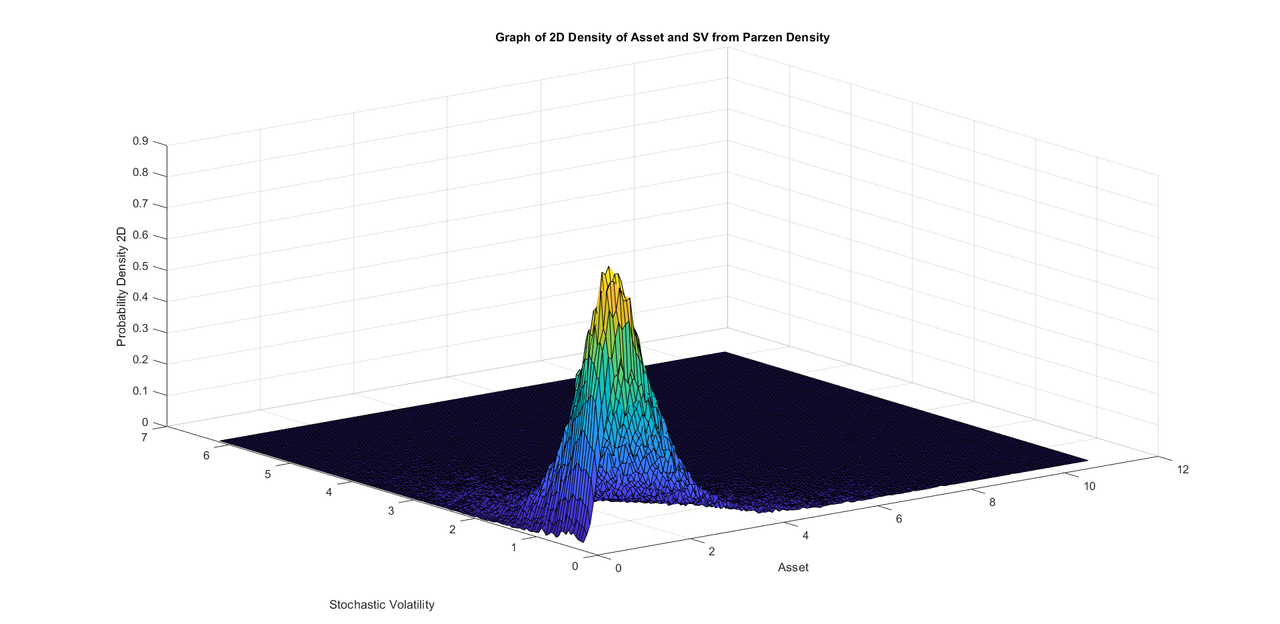.
..
.
.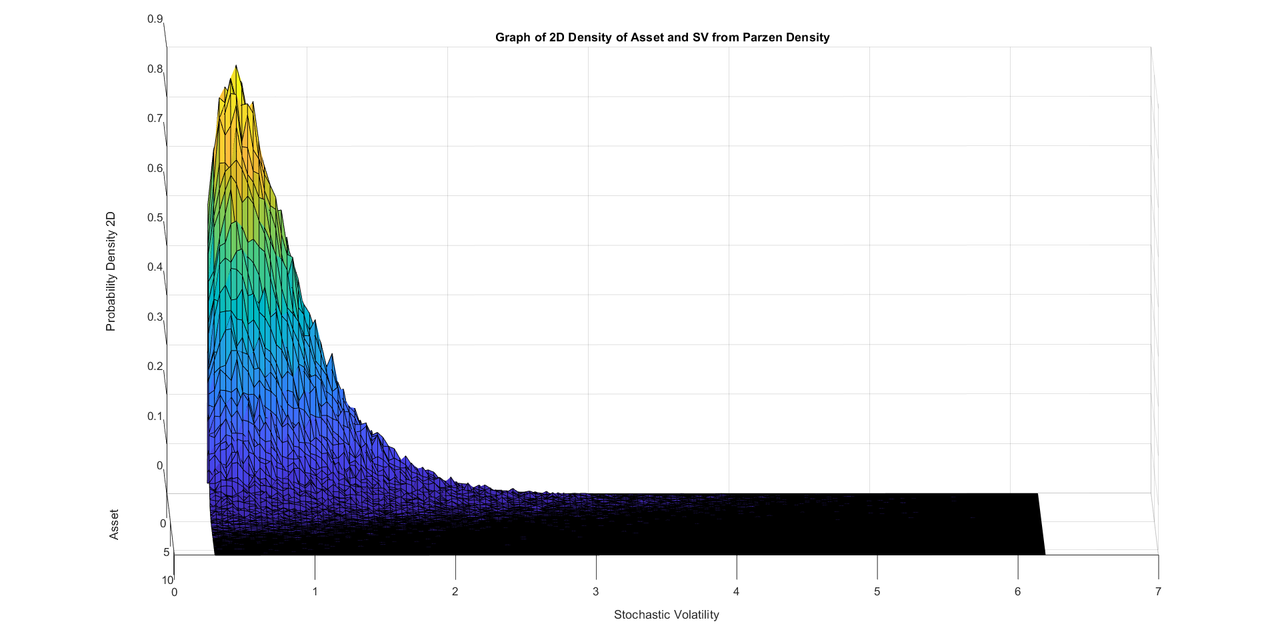.
.
.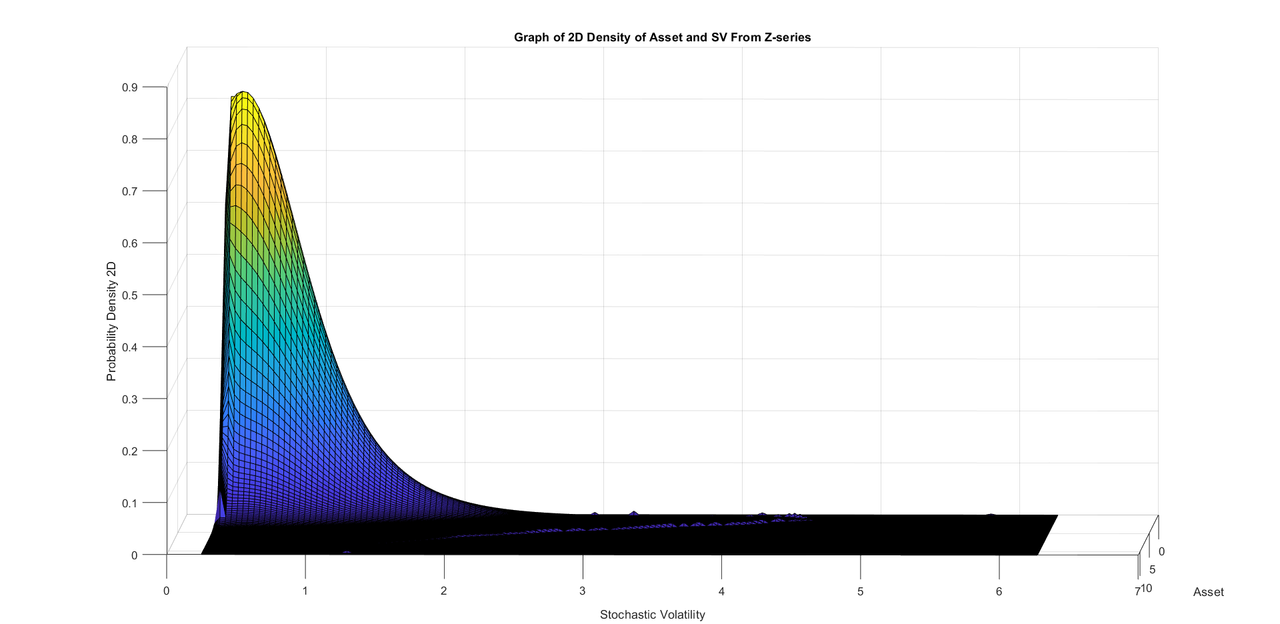.
.
.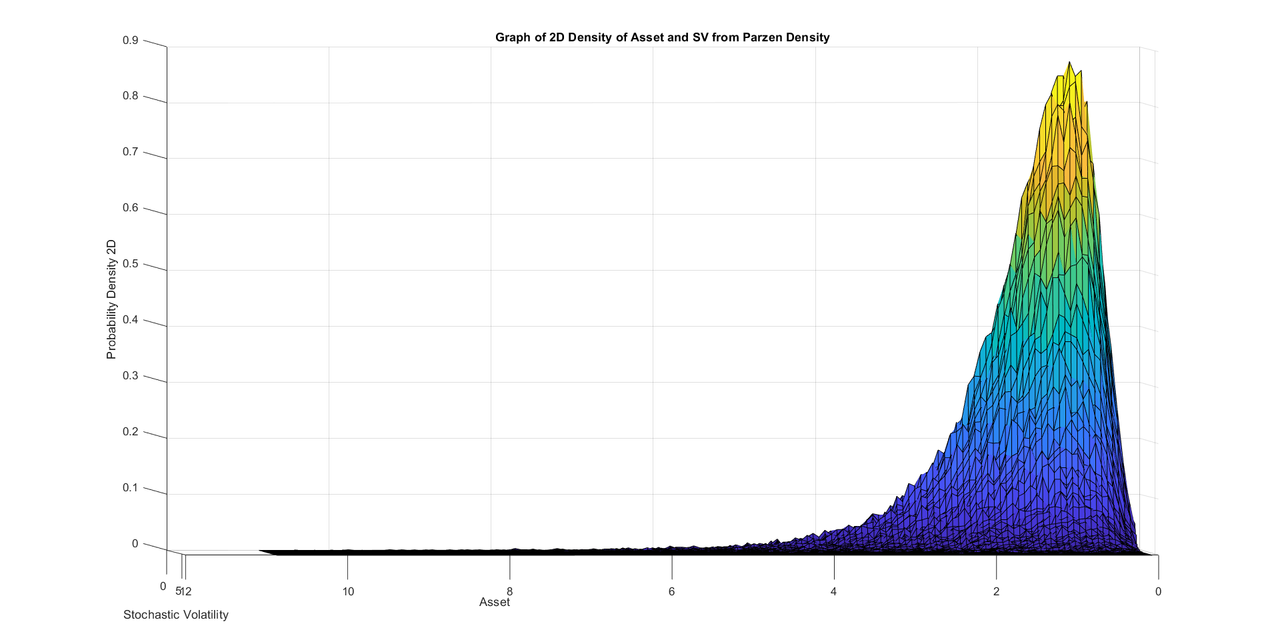.
.
.
[url=https://postimg.cc/zVdPNMxj]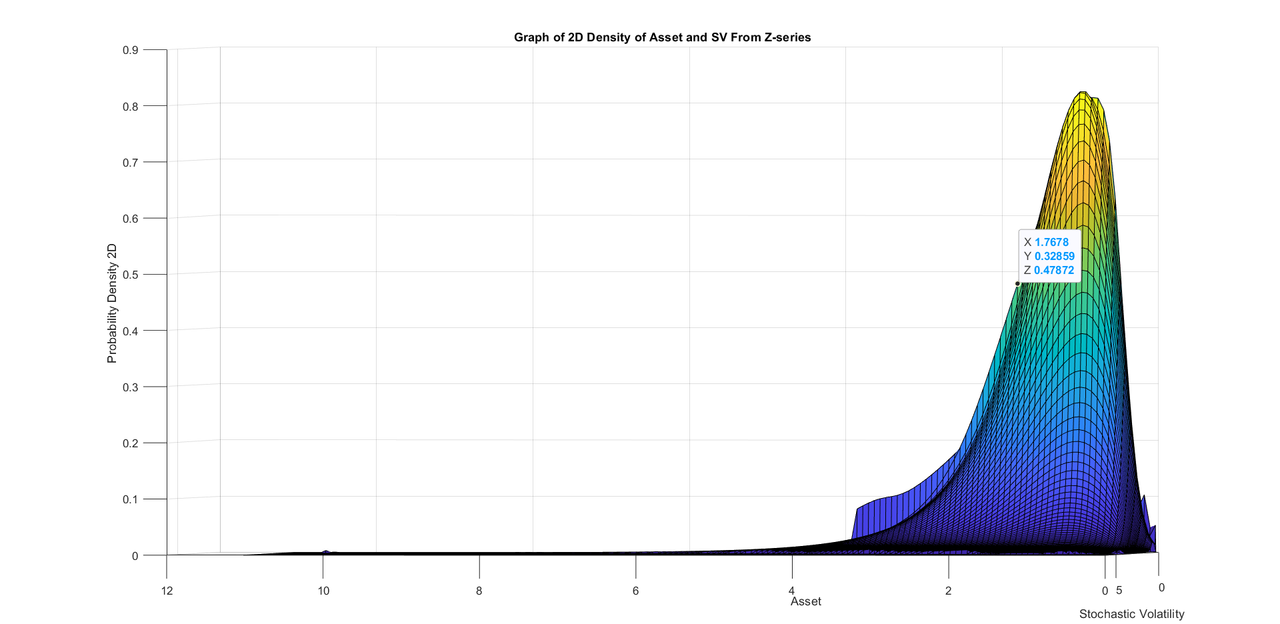[/url]
.
.
.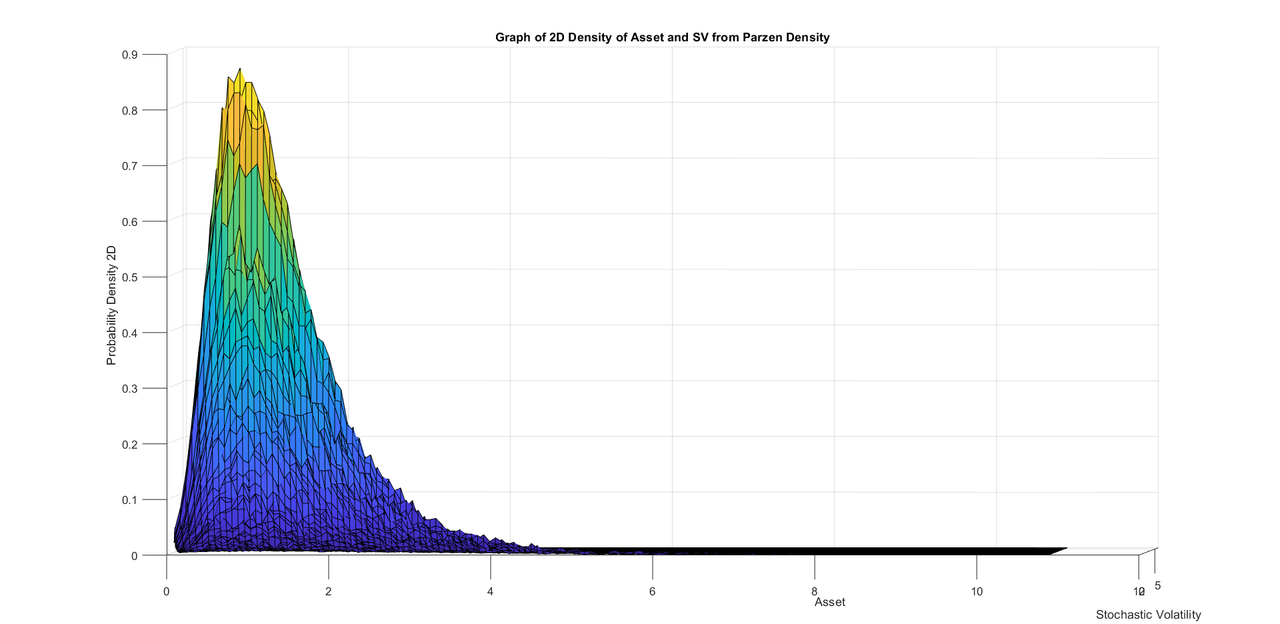.
.
.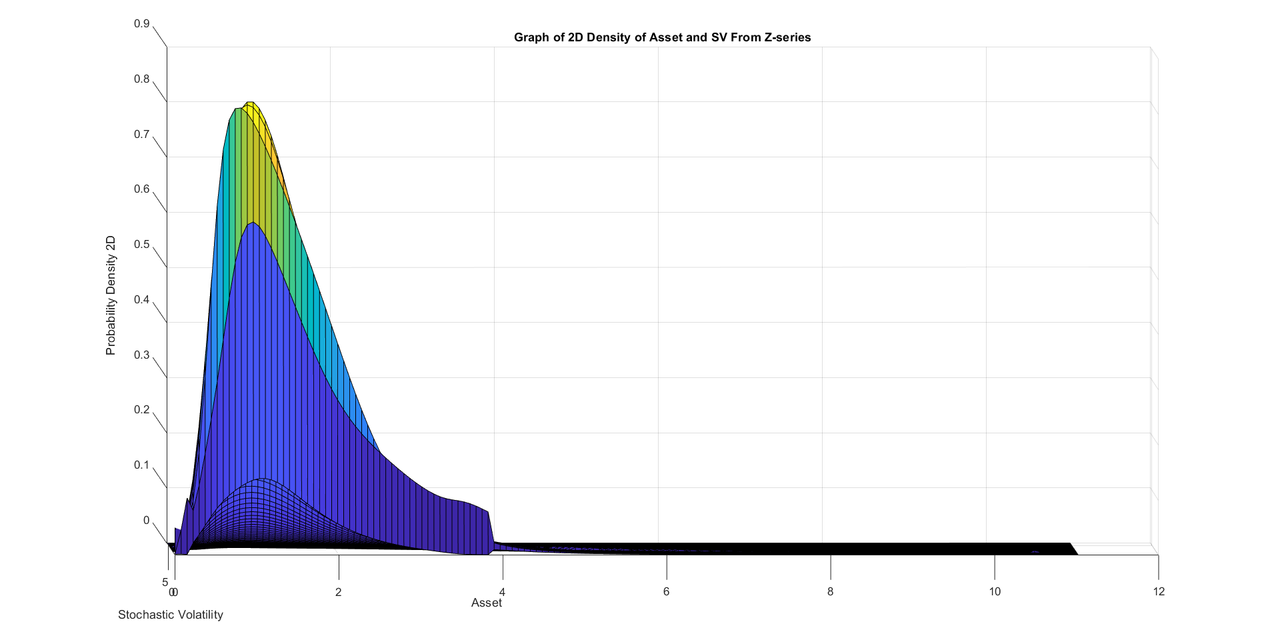Last edited by Amin on September 22nd, 2023, 10:22 am, edited 1 time in total.
You think life is a secret, Life is only love of flying, It has seen many ups and downs, But it likes travel more than the destination. Allama IqbalAmin
Topic Author
Posts: 2416
Joined: July 14th, 2002, 3:00 am

### Re: Breakthrough in the theory of stochastic differential equations and their simulation

Friends, even though I was able to create initial graphs, I am still afraid that there is a small issue with my algorithm that is slightly putting off the density. I think I know the problem and I am trying to investigate it and see how to fix it. I hope to be able to settle it soon and them come back with explanation of the algorithm and the matlab programs.
You think life is a secret, Life is only love of flying, It has seen many ups and downs, But it likes travel more than the destination. Allama IqbalAmin
Topic Author
Posts: 2416
Joined: July 14th, 2002, 3:00 am

### Re: Breakthrough in the theory of stochastic differential equations and their simulation

Friends, I am posting some new graphs for another SV monte carlo run. I was thinking that my analytic density is slightly shifted lower than the numerical density whenever I would make a comparison. Then I thought of making a surface plot of differences in the 2D probability functions of between numerical density and the analytic density as both densities are calculated exactly on the same coordinates grid. After looking at the 2d surface plot of differences in probability function, I notice that they are almost equally distributed on both sides of the Z-axis. I have given all these graphs of difference of  probability density with different angles of the 2D density. I think my 2D inversion of probability density function algorithm is quite reasonable and I will be posting it tomorrow.
My new laptop computer was extremely helpful at just the right time otherwise I would not have been able to complete this work properly.
I will try posting several 2D graphs in later posts with different SDE parameters. Parameters of the SDE in this case are given after the graphs.

.
.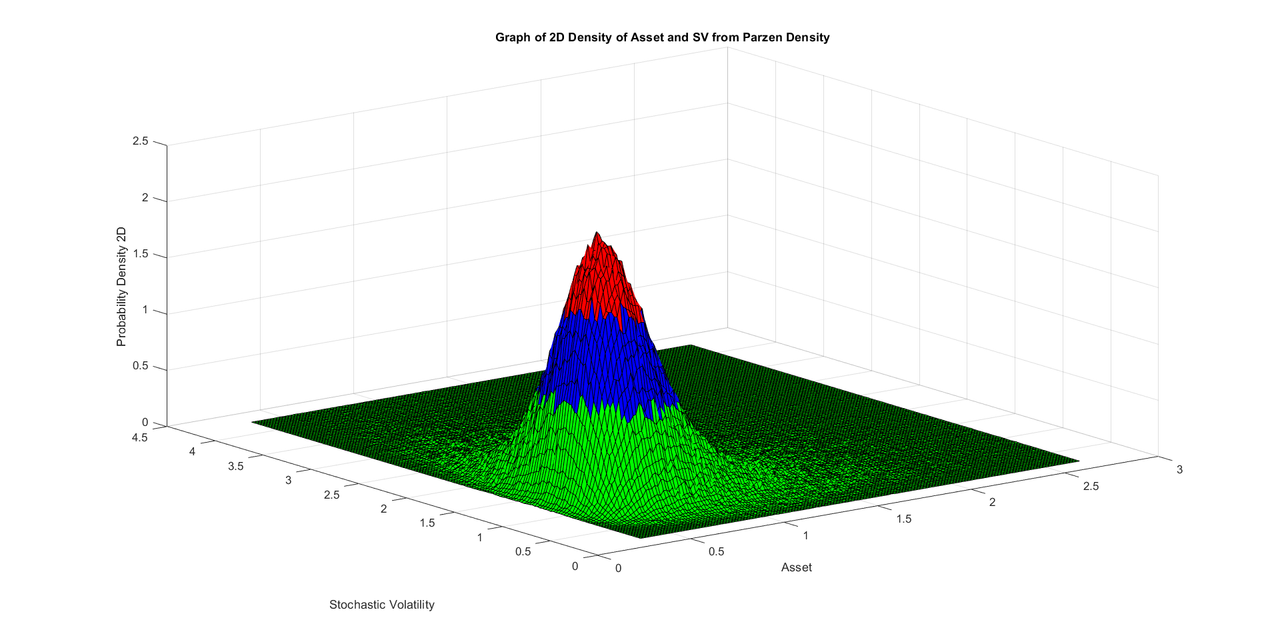.
.
.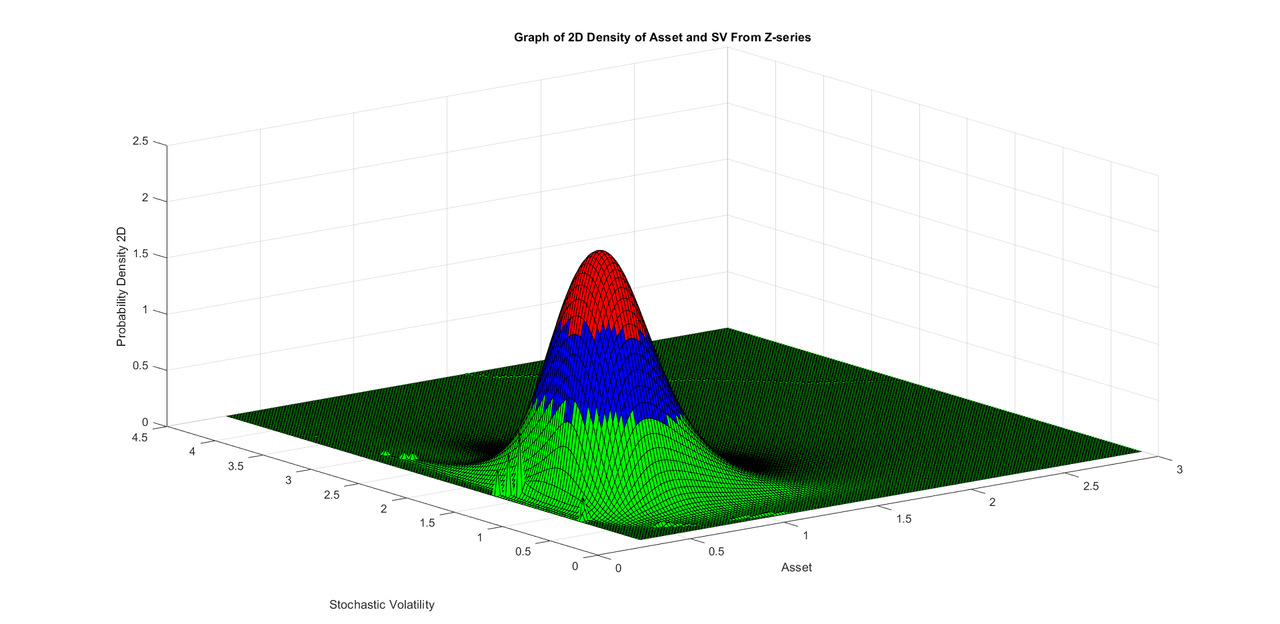.
.
.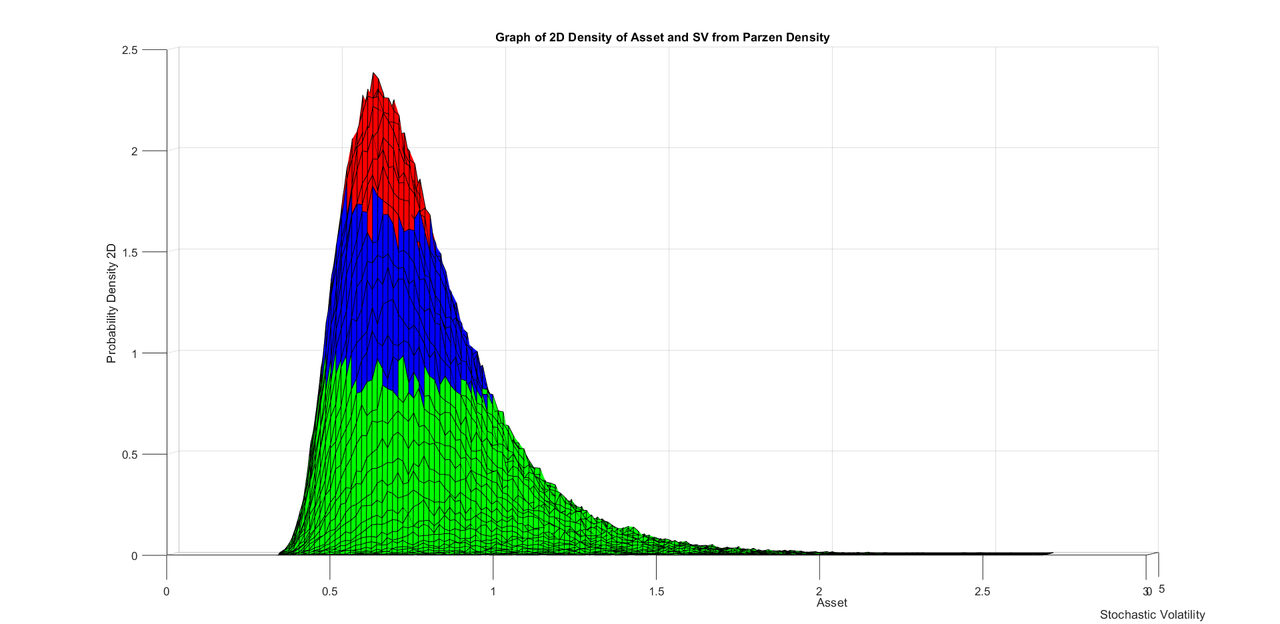.
.
.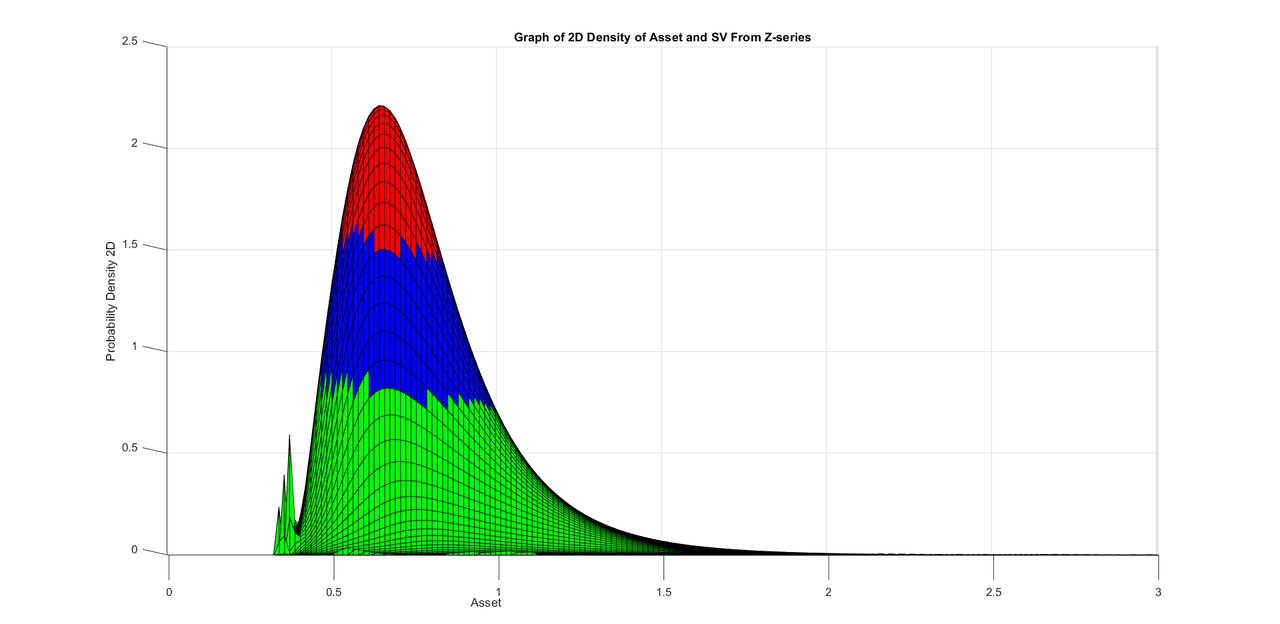.
.
.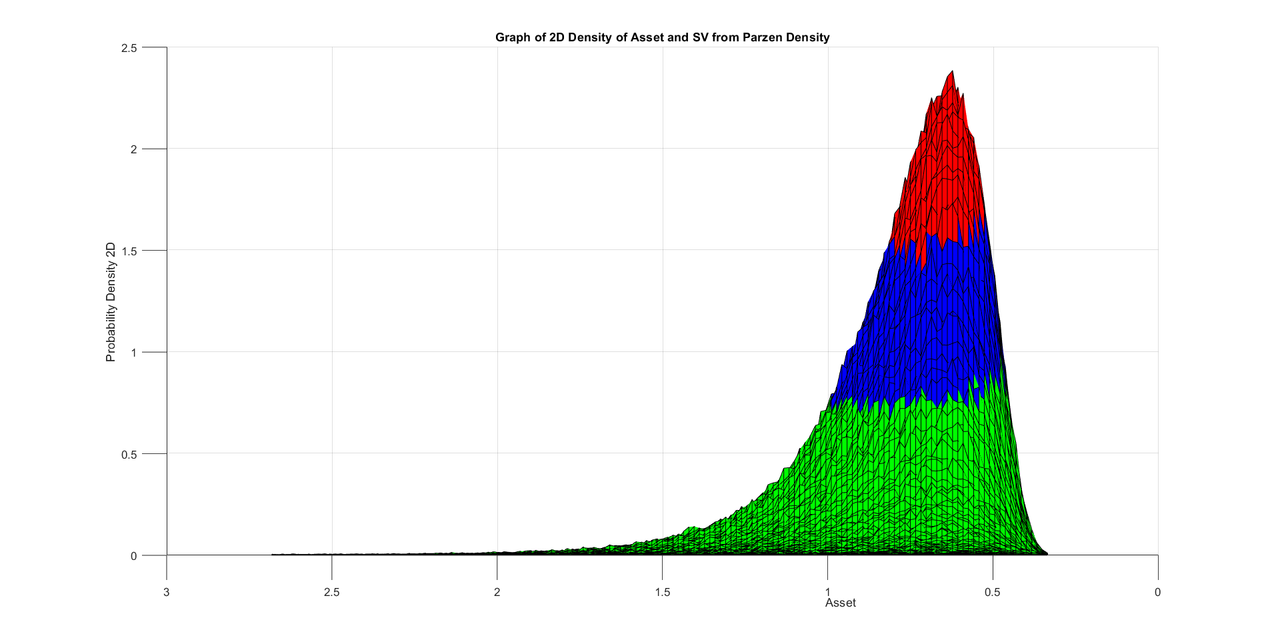.
.
.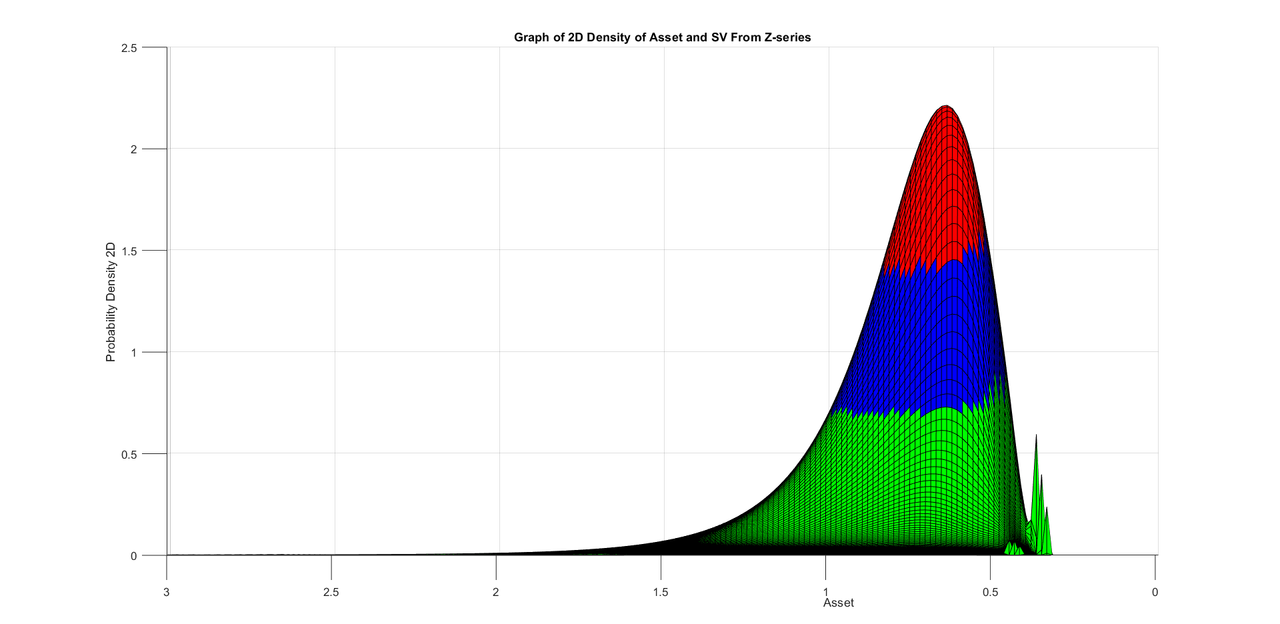.
.
.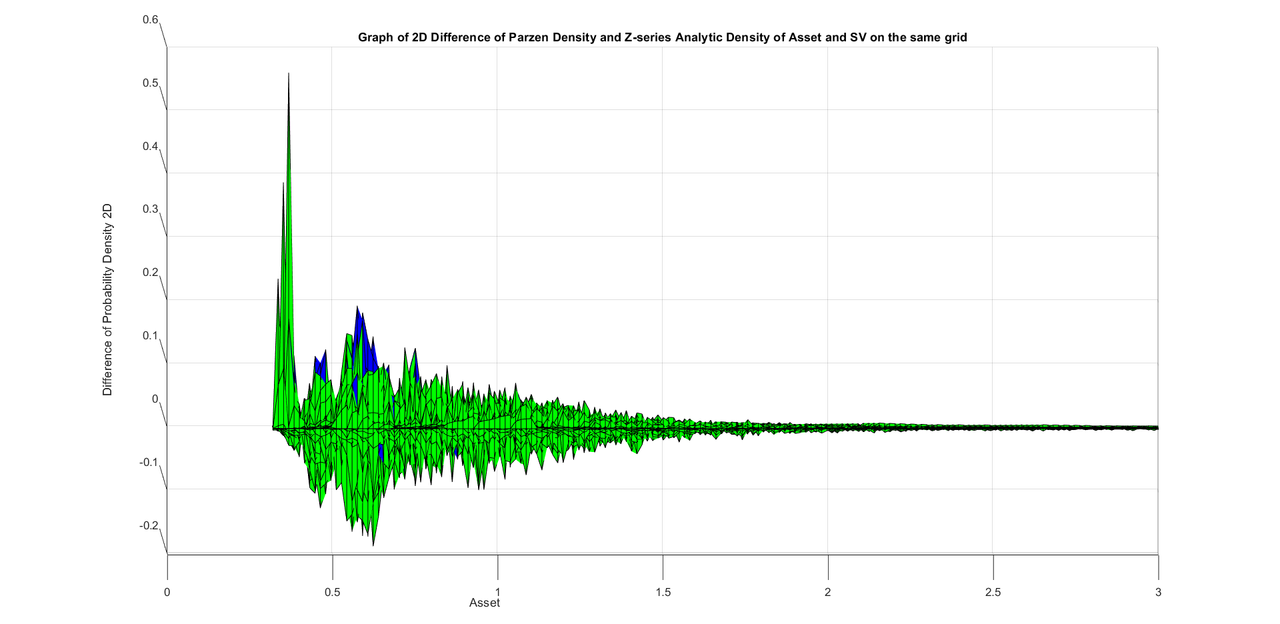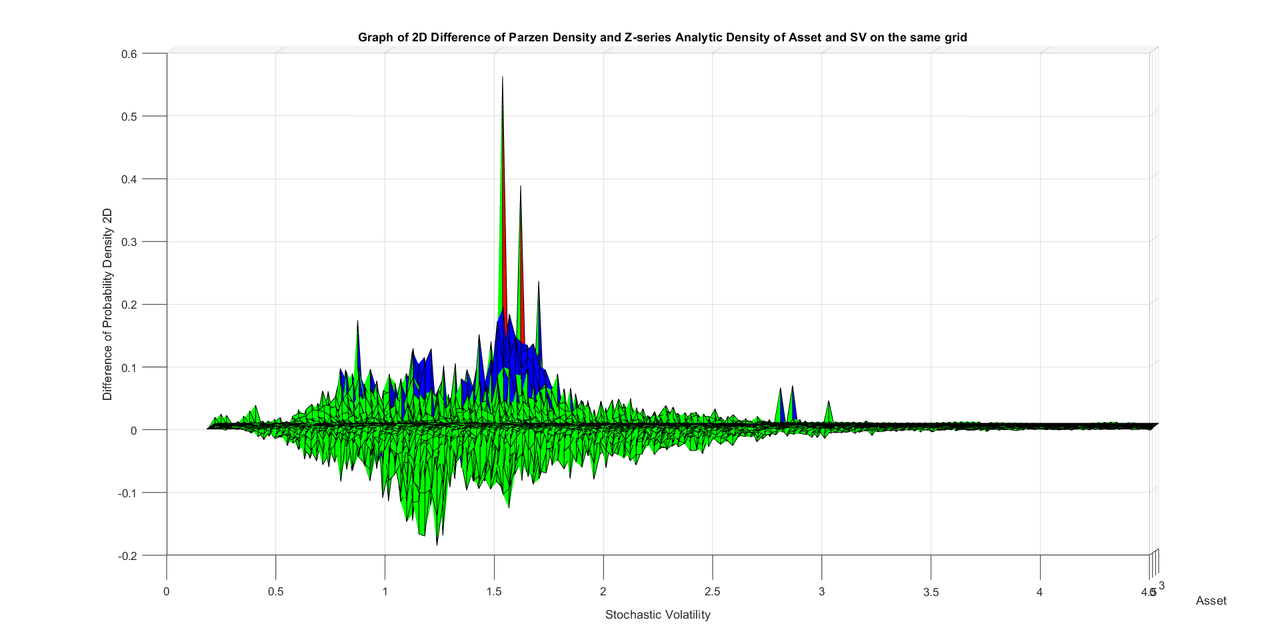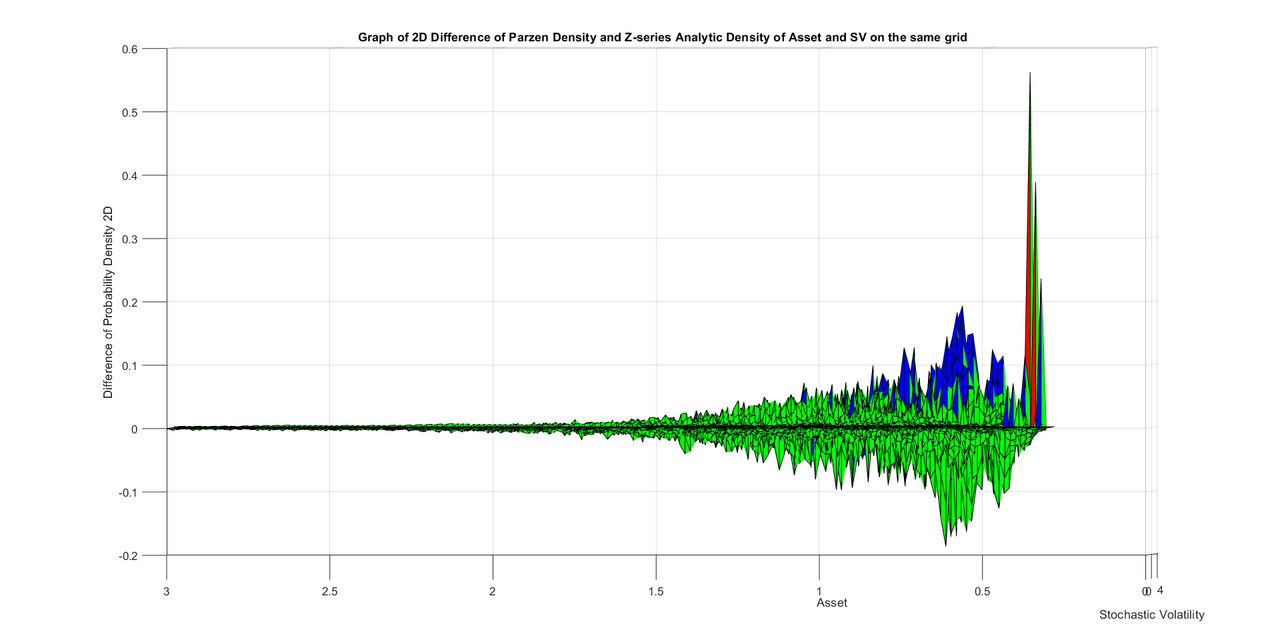.
.
.
SDE parameters for the monte carlo are given below

X0=1.0;
alpha1=0;
alpha2=1;
kappaX=1;
thetaX=1.0;
a=kappaX*thetaX;
b=-kappaX;

rho=0;
sigma1=.35;
gammaV=.5;
gammaX=.95;
%%%%%%%%%%%%%
V0=1.0;
thetaV=1.0;
kappaV=1.750;
mu1=kappaV*thetaV;
mu2=-kappaV;
beta1=0;
beta2=1;
gamma=.85;
sigma0=.8;
%%%%%%%%%%%%

dt=.03125;
Tt=32*4;

T=Tt*dt;

%%%%%%%%%%%%%%%%%%%%%%%%5
You think life is a secret, Life is only love of flying, It has seen many ups and downs, But it likes travel more than the destination. Allama IqbalAmin
Topic Author
Posts: 2416
Joined: July 14th, 2002, 3:00 am

### Re: Breakthrough in the theory of stochastic differential equations and their simulation

I noticed that asset in previous post was mean reverting. These are SDEs with same parameters but asset has no mean reversion. I will give the SDE parameters at the end.
I have captured the original graphs and angles from all four sides.
.
.
.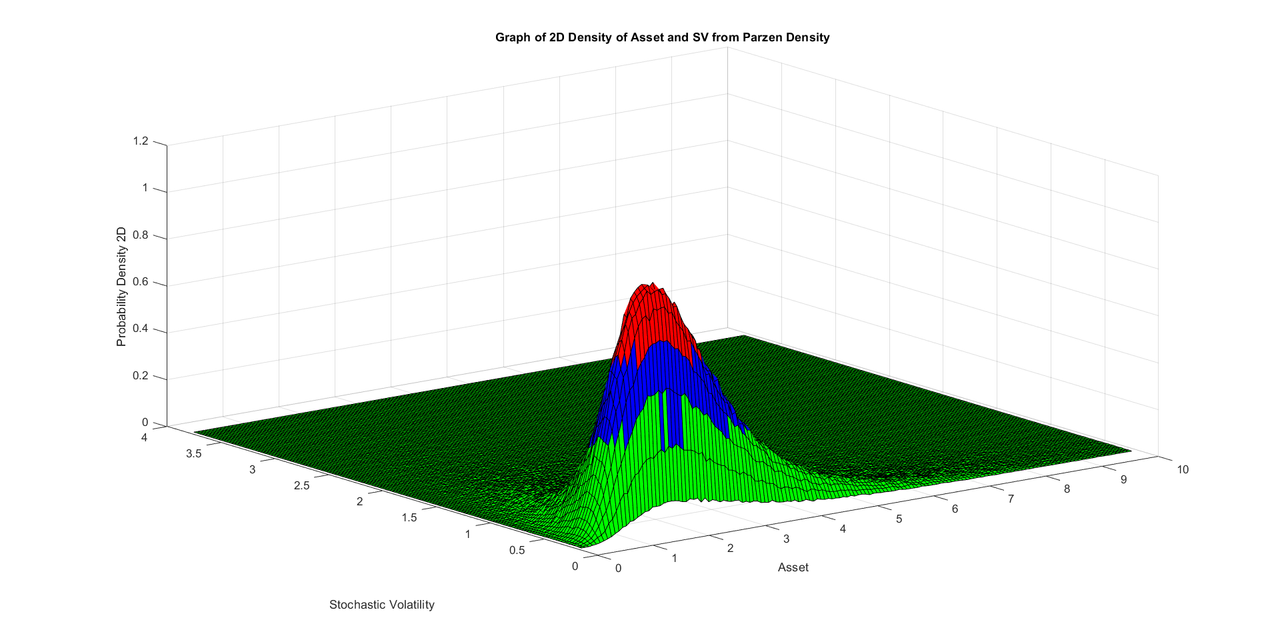.
.
.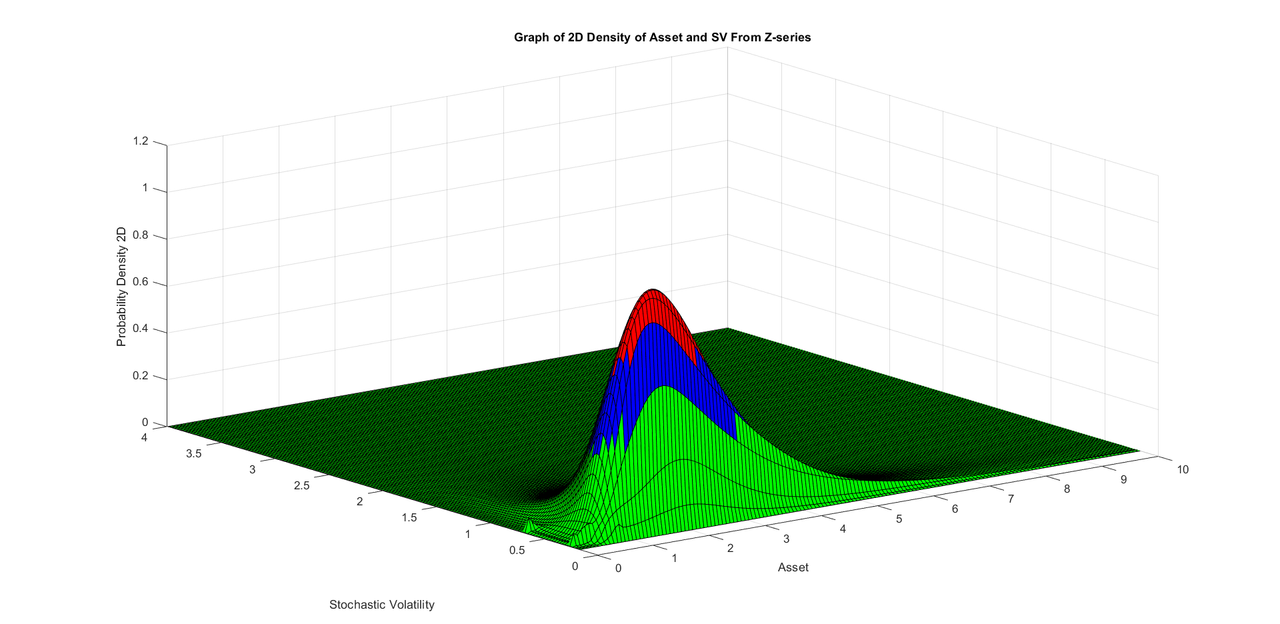.
.
.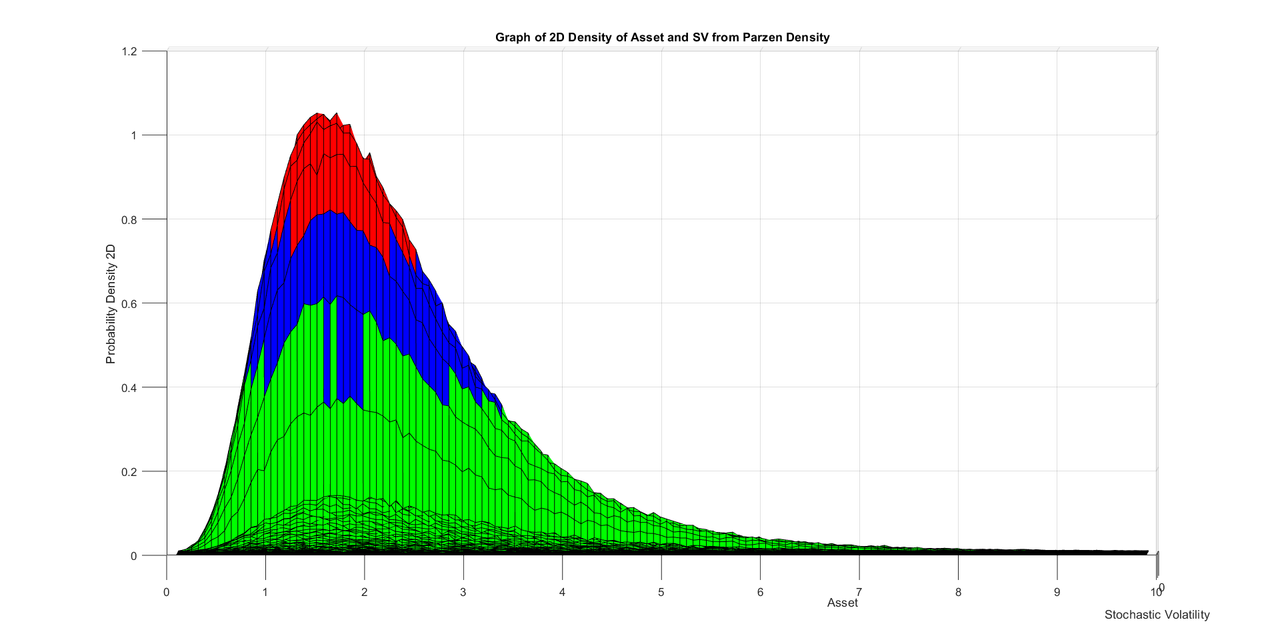.
.
.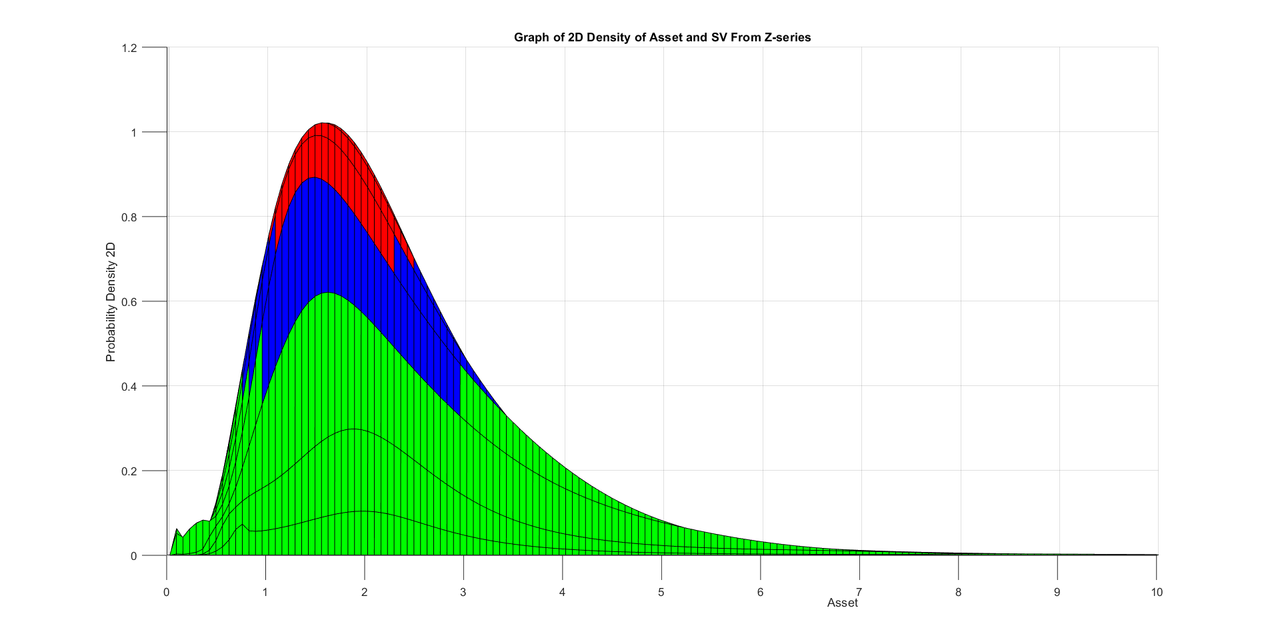.
.
.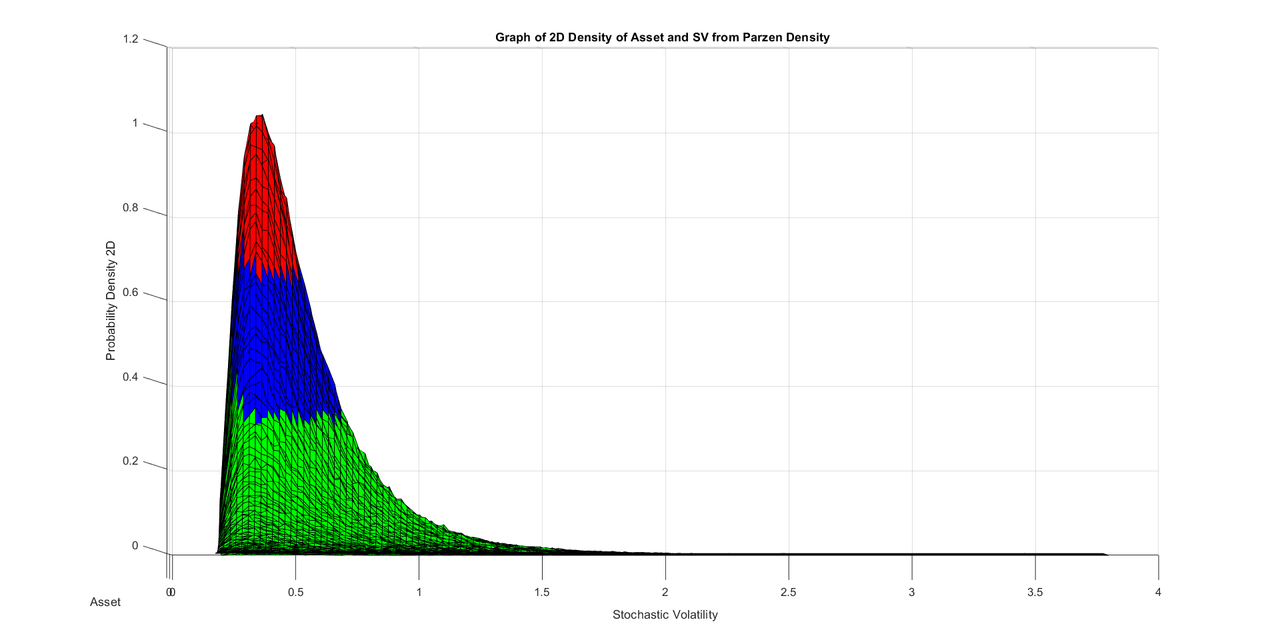.
.
.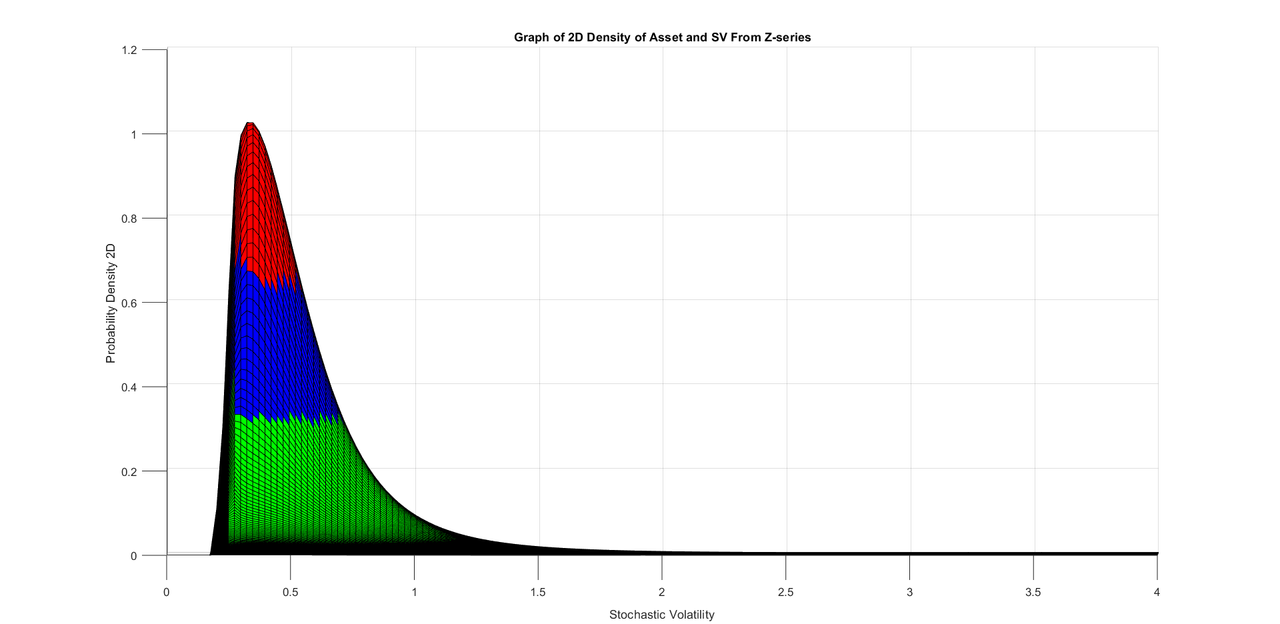.
.
.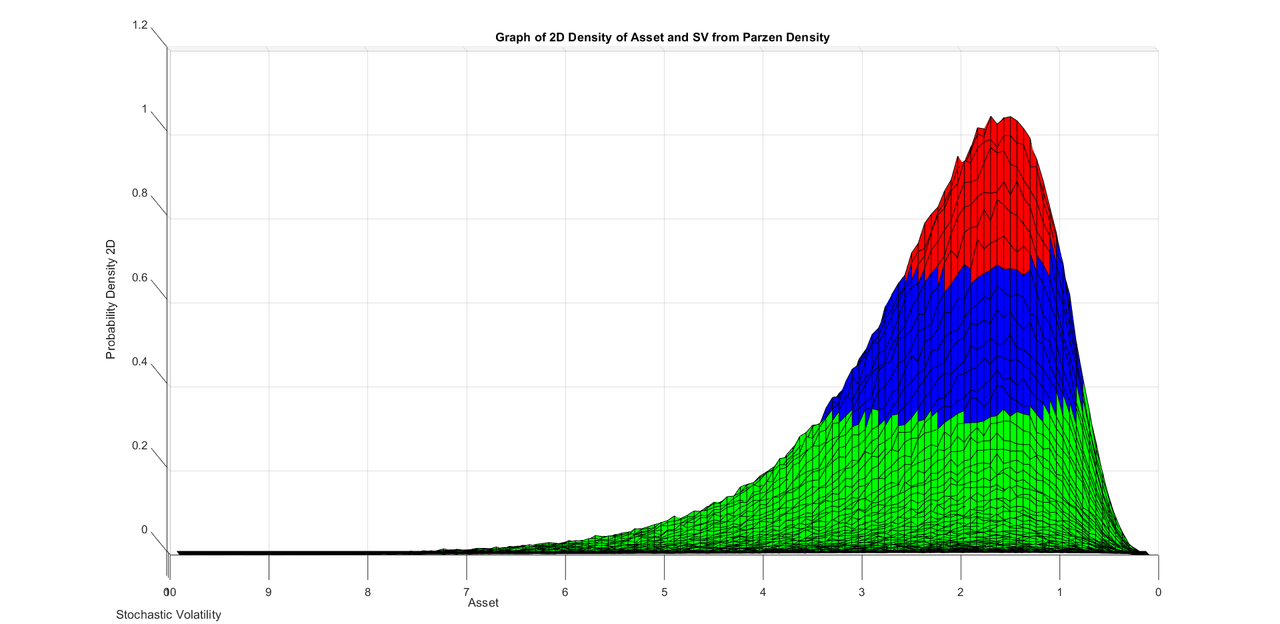.
.
.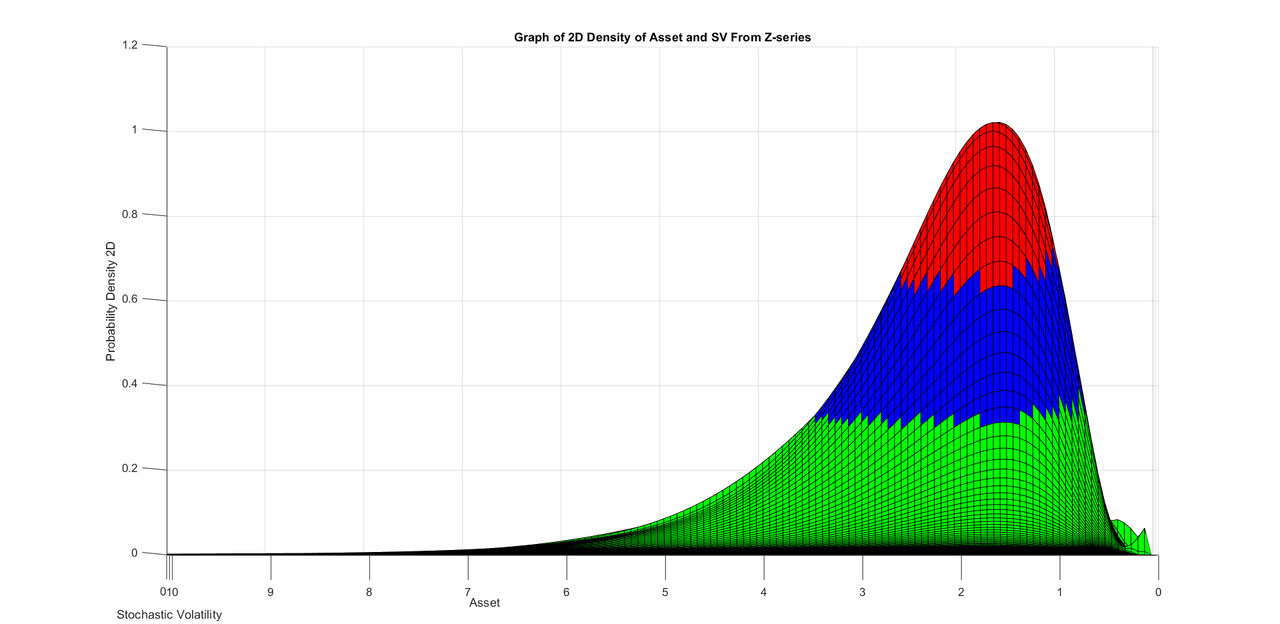.
.
.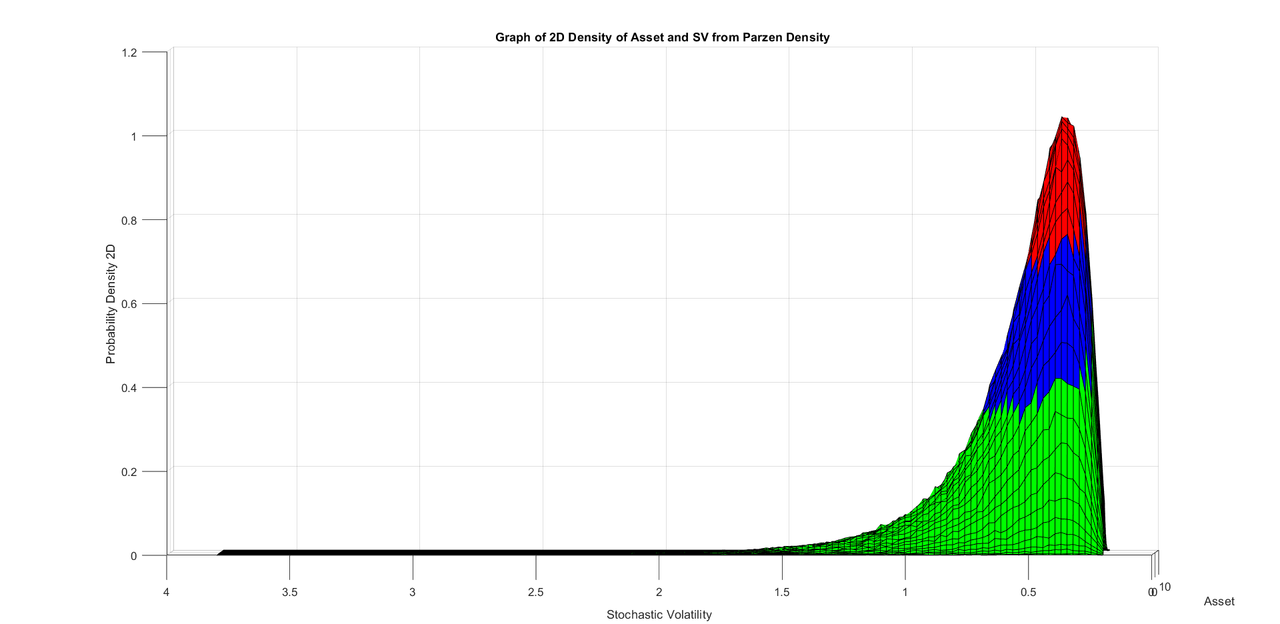.
.
.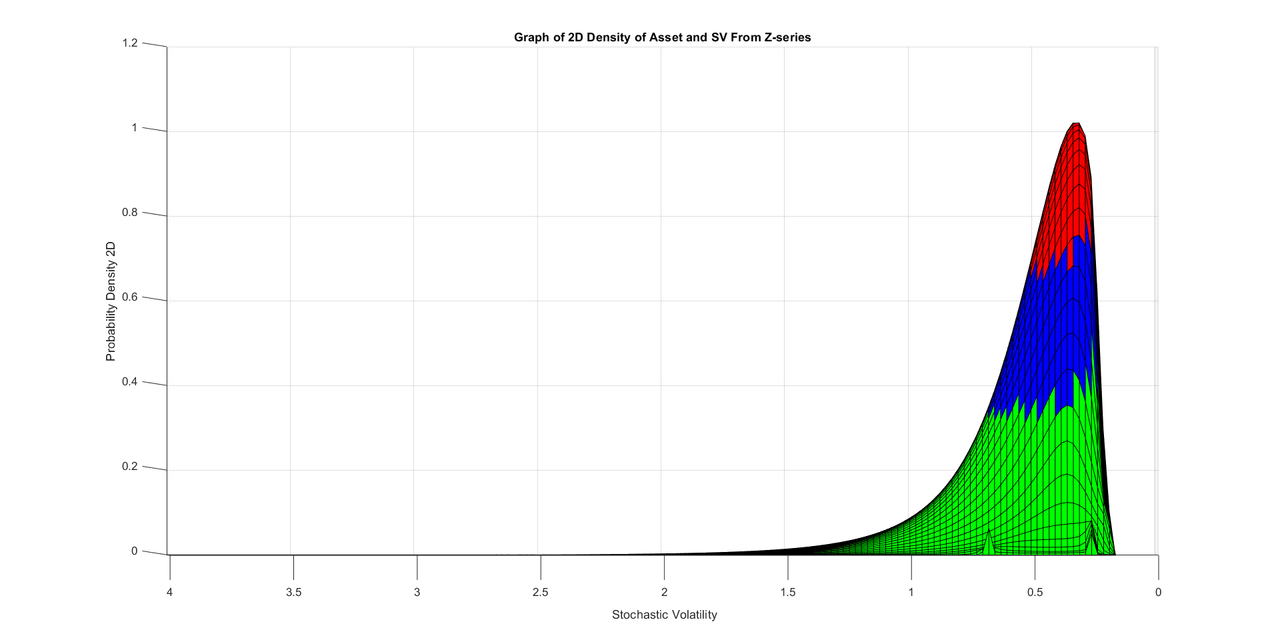.
.
.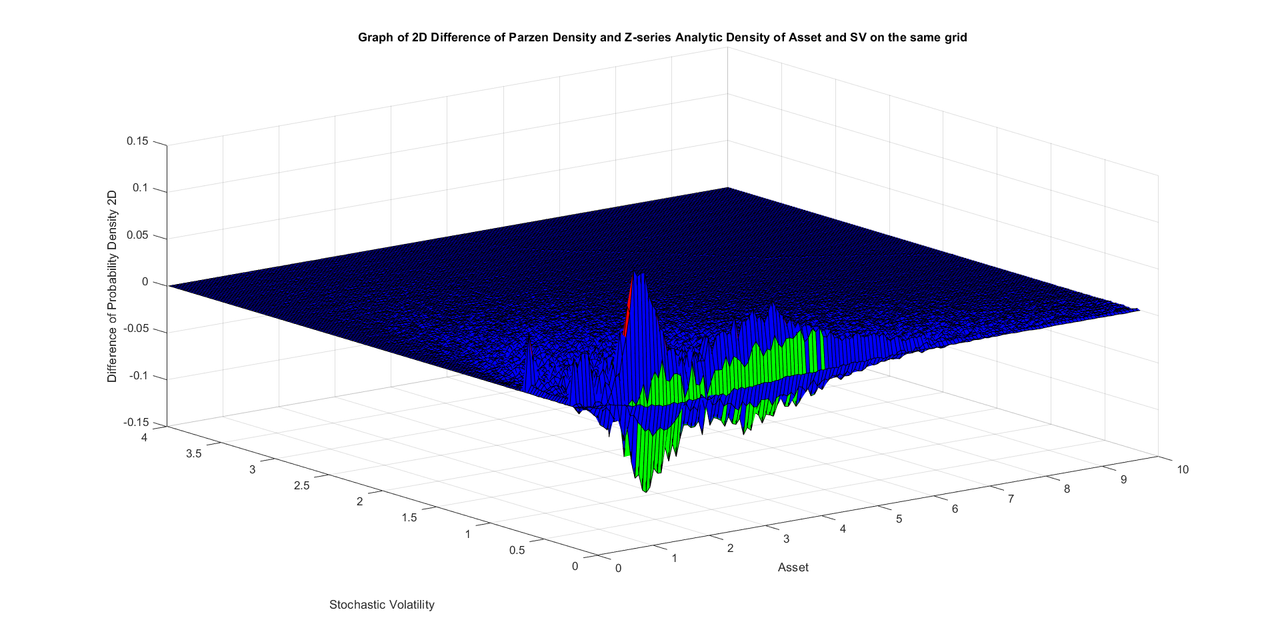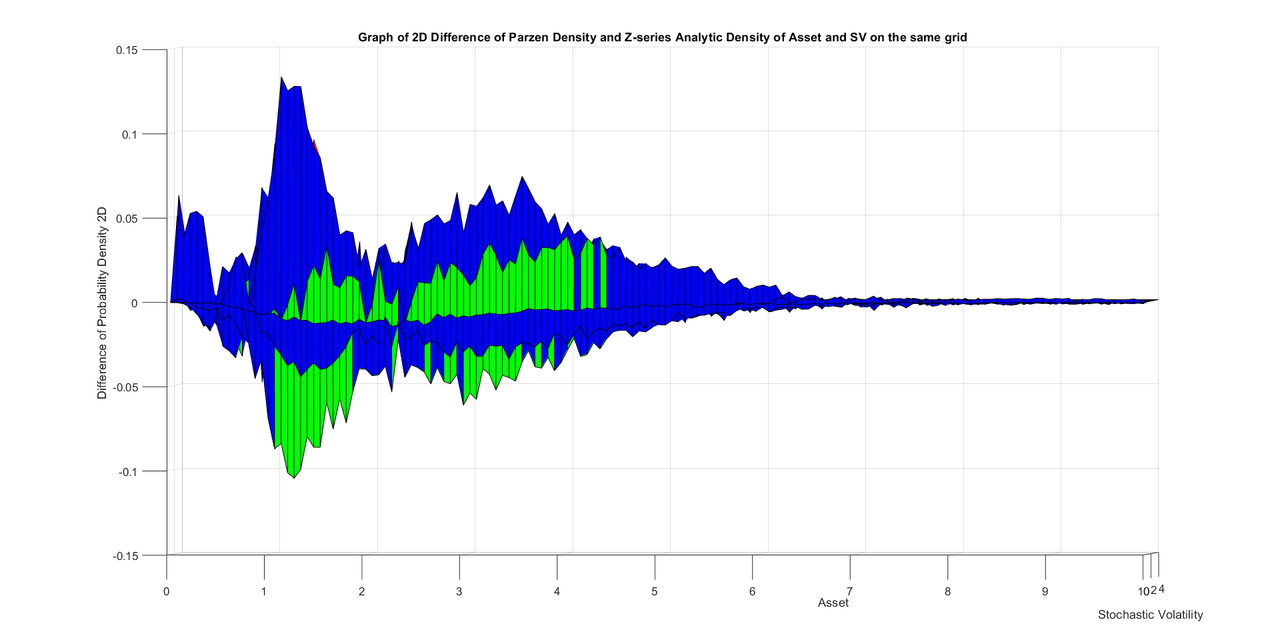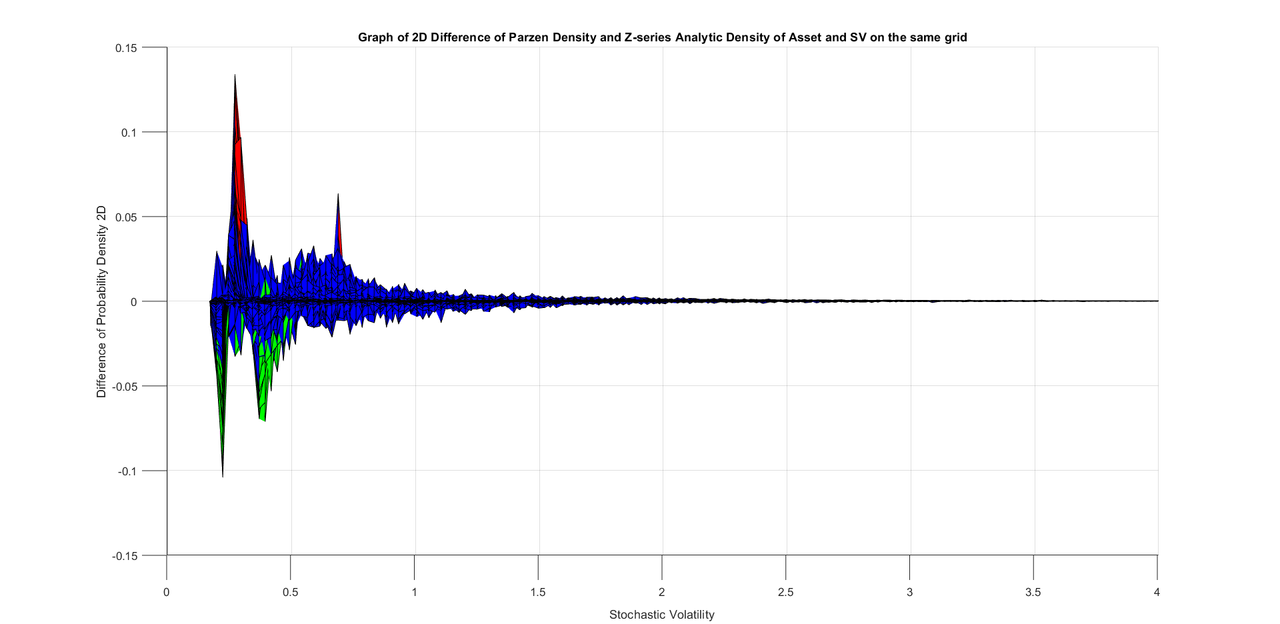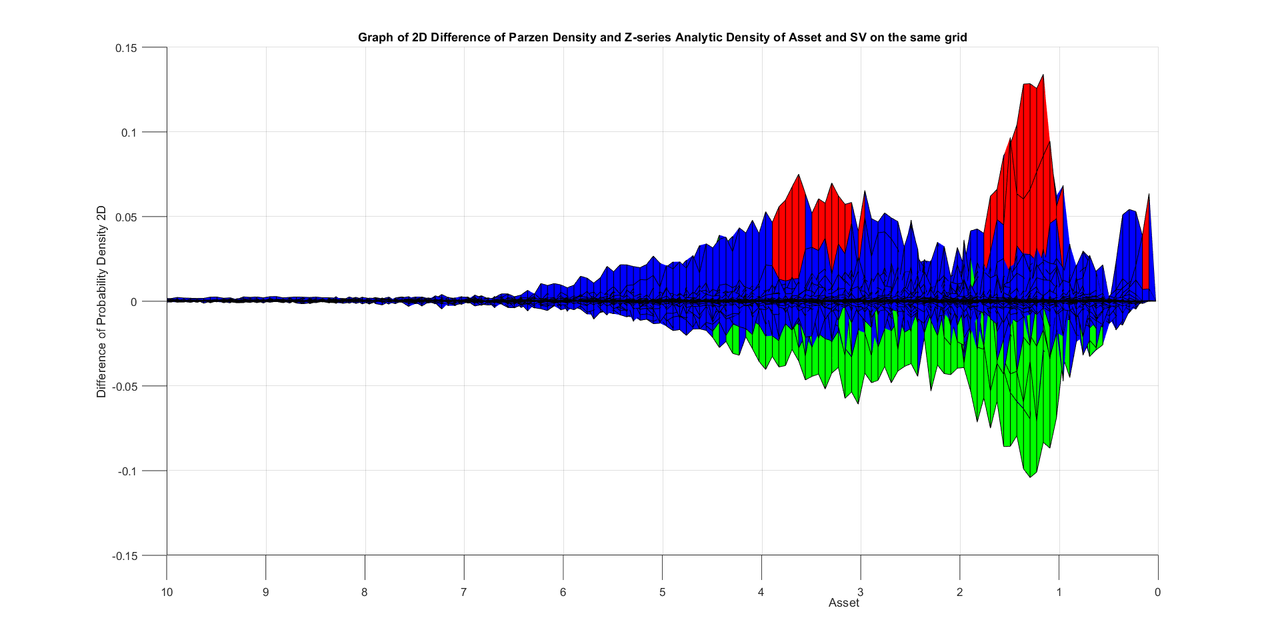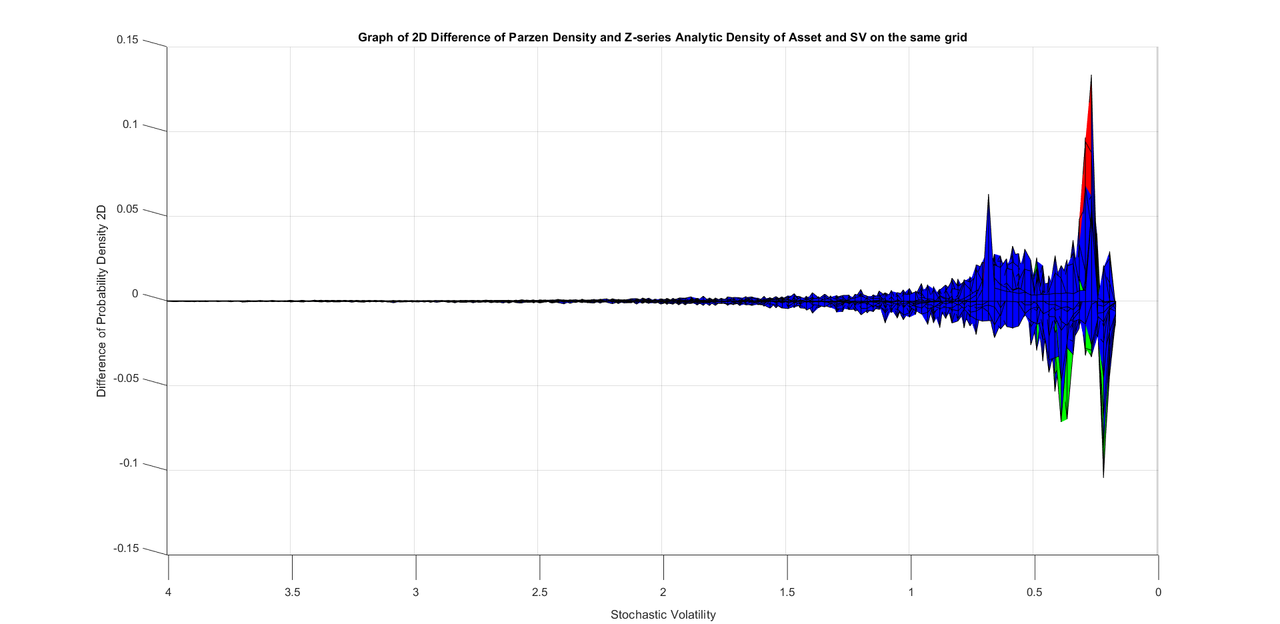.
.
.
Here  are parameters of the SDEs used in monte carlo.

X0=1.0;
alpha1=0;
alpha2=1;
kappaX=0;
thetaX=0.0;
a=kappaX*thetaX;
b=-kappaX;

rho=0;
sigma1=.35;
gammaV=.5;
gammaX=.95;
%%%%%%%%%%%%%
V0=1.0;
thetaV=1.0;
kappaV=1.750;
mu1=kappaV*thetaV;
mu2=-kappaV;
beta1=0;
beta2=1;
gamma=.85;
sigma0=.8;
%%%%%%%%%%%%

dt=.03125;
Tt=32*4;

T=Tt*dt;

%%%%%%%%%%%%%%%%%%%%%%%%5
You think life is a secret, Life is only love of flying, It has seen many ups and downs, But it likes travel more than the destination. Allama IqbalAmin
Topic Author
Posts: 2416
Joined: July 14th, 2002, 3:00 am

### Re: Breakthrough in the theory of stochastic differential equations and their simulation

Friends, I am working to write comments on the new matlab program to find 2D Hermite Series from of 2D random variables from monte carlo in SV setting. I hope to post the program in a few hours. I will also try to write a post that explains my inversion of 2D Hermite series to find the bivariate probability density on the 2D specified grid.
These are initial programs and work need to be done to settle all the issues for example in SV setting, I noticed in many SDEs when vol of vol gets close to one or is above one, the match between Hermite Series density and numerical density decreases. I think this can be improved by adding another two hermite polynomials in each direction. Since we find our 2D polynomial coefficients by inner product method, the numerical complexity does not increase much as we increase the order of the series somewhat (as opposed to optimization to find the hermite series from moments). We will also have to tackle grid issues since on a uniform grid Z changes by a very large amount when X<1 and V<1 since most probability mass is concentrated here ( and on a large uniform grid relatively very few grid points are within in this area so far lesser sampling where most of the density is concentrated) and changes very very slowly when X and V are large. I really think that designing a better non-uniform grid will greatly improve the quality of inner product calculation of 2D series coefficients. Current program is very basic and I hope we will continue to work on the problem for coming weeks and months to be able to properly  calculate 2D Coefficients in all SDE scenarios and later also do research on 2D regressions of monte carlo pay off on the asset and vol in order to be able to find better greeks and do better hedging.
Sometimes, for accuracy in mote carlo, you might have to decrease the time step size when stochastic volatility and vol of vol are quite high.
As a next step, I plan to calculate numerical 2D monte carlo density on a non-uniform grid with a fast algorithm that just requires one division per monte carlo path just like we had for uniform 2D grid. Also inversion of 2D hermite series on non-uniform grid does not require any more effort and we could use the same algorithm. I will also see if we need a higher order of hermite series in difficult SV problems and add that capability if the program if we need that.
There might also be some possible minor errors in the program but I am sure we will slowly move towards a better understanding of the problems involved and far perfect programs for risk management.
You think life is a secret, Life is only love of flying, It has seen many ups and downs, But it likes travel more than the destination. Allama IqbalAmin
Topic Author
Posts: 2416
Joined: July 14th, 2002, 3:00 am

### Re: Breakthrough in the theory of stochastic differential equations and their simulation

Friends, mind control agents are altering my matlab programs I am working on. I am getting all statistics right with monte carlo but results of my 2D numerical density and related graphs are many times totally absurd. I cahanged everything that could go wrong but to no avail.
They used to change/alter my programs on a daily basis around 2013-2015 and I used to mention that on the forum and probably some good people protested and they stopped altering my programs on a daily basis. This is from before I had done any significant research.
I had told friends that I have very good market trading algorithms and I tested them several times on different data with excellent results. A few months ago I wanted to actually try the algorithms on Binance. My Brother-in-law had said that he would finance any money to run the algorithms and for operational costs. However, when I tried the very recent Binance data at that time, I noticed that my algorithms had sharply reduced profitability. I really thought at that time that crypto market had changed its dynamics and my algorithms would no longer work. I was slightly embarassed before my brother-in-law but told him that I have to further improve my trading algorithms. I still had all the data and kept it on my computer. And about two months ago, out of the blue without any previous planning or thought, I just tried very same algorithms on the data from the time with little profitability and I was surprised to see that my results were actually very good. I realized that they had altered my programs when I needed them the most.
There are other times I know when I was very sure that they altered my programs in past two years even though it was very rare and this happened for just a few occasions.
They have total control of my computers. And I think they do not physically alter the code on my computer, but when I write a command to run a certain file they just replace their file with my file and what actually runs on computer comes from their file. They somehow make sure that my commands are replaced with their commands and their code runs on my computer.
I had told friends that I was earlier working on an old computer from 2012. They again had complete control of the computer. They even had control whether computer would turn on with electricity or not and they could also shut it down abruptly. The extension wire I used with computer had a loose socket but it was not a big deal, I would adjust the computer plug in the socket and play with it for 10-20 seconds and the computer would show that it is attached to electric power. However, one day I continued to try for twenty minutes to turn on the computer but it would not turn on and electricity light on it would not even blink at all. I was totally perplexed what was wrong. Frustrated, I took an electric tester that shows red light when it is attached to electric current. When I attached the tester to the side of computer wire that goes into the computer, it started to show red light. Power was actually going into the computer. When they realized that I had noticed that electric current is going into the computer, they enabled the electricity and the computer turned on the very minute. I had continued to try for twenty minutes but the computer would just not turn on despite that electricity was going into the computer.
So they have total absolute control of my computers.
When I bought this thinkpad, a few days ago, I started using it on the same program on the new computer and I was so happy that it worked very fast and I also mentioned it on internet. But after two days, they did something and speed of the computer remarkably decreased on my programs. They try to do everything to thwart me.
Though American army had been good enough to give me concessions to not alter my programs and in so many other ways when good American people protested to them but now they strongly regret that they made it easier for me to do any research under pressure from good people. So now whenever they find opportunity, they try to thwart me in some way they can.
They are again changing/altering my programs since they completely hate that I get any limelight when trying to do good research. It helps my human cause to end cruel mind control everywhere( as people understand the true nature of mind control torture and reasons behind it )for which I have been fighting for more than a decade and corrupt officers in American army are afraid once mind control ends, their livelihood due to corruption and obliging rich people by retarding innocent and intelligent humans would completely end.
I hope good American friends protest to American army and ask them to not alter my programs and I will be able to post my programs after that.
I will be making a post explaining how I inverted the 2D Hermite Series to get the density. I will also post the function that inverts the 2D hermite series to find the 2D density in a bit but I cannot post all the matlab program until I am sure it works well. I hope once people pressurize American army crooks to end altering my programs, I will post them right away. I really look forward to posting my programs on Wilmott as I have always done before.
You think life is a secret, Life is only love of flying, It has seen many ups and downs, But it likes travel more than the destination. Allama IqbalAmin
Topic Author
Posts: 2416
Joined: July 14th, 2002, 3:00 am

### Re: Breakthrough in the theory of stochastic differential equations and their simulation

This is the program I used for inversion of 2D Hermite Series to get the 2D probability density. I will be explaining it in the next post.
.
.
.
function [pXY2D] = Calculate2VariablesOn2DGridFrom2DZSeries(XX,YY,NoOfBinsX,NoOfBinsY,C,a0,a,BinSizeX,BinSizeY)

%YY are Y-coordinates of 2D Grid. In our case this is stochastic
%volatility and is univariate and independent of asset so it can be
%inverted as a univariate Z-series. its Z-series is given by a0 and a.

[Zy] = CalculateZgivenXAndZSeriesC7(YY,a0,a);

for mm=1:NoOfBinsY+1
if(Zy(mm)>5.0)
Zy(mm)=5.0;
end
if(Zy(mm)<-5.0)
Zy(mm)=-5.0;
end
end
Zy

str=input("Look at Zy");

bH(1:NoOfBinsY+1,1:7)=0;
bH0(1:NoOfBinsY+1)=0;
for mm=1:NoOfBinsY+1
%bH0(mm)=C(1,1);
for Ovm=1:8
for Oxn=2:8

bH(mm,Oxn-1)=bH(mm,Oxn-1)+C(Oxn,Ovm).*HermiteH(Ovm-1,Zy(mm));

end

bH0(mm)=bH0(mm)+C(1,Ovm).*HermiteH(Ovm-1,Zy(mm));
end
end

for mm=1:NoOfBinsY+1
bH0(mm)=bH0(mm)./YY(mm);
for Oxn=1:7
bH(mm,Oxn)=bH(mm,Oxn)./YY(mm);
end
end

for mm=1:NoOfBinsY+1
[b0,b]=ConvertHCoeffsToZCoeffs(bH0(mm),bH(mm,1:7),7);
[Zx0] = CalculateZgivenXAndZSeriesC7(XX,b0,b);
Zx(1:NoOfBinsX+1,mm)=Zx0(1:NoOfBinsX+1);
end

for mm=1:NoOfBinsY+1
for nn=1:NoOfBinsX
if(Zx(nn,mm)>5.0)
Zx(nn,mm)=5.0;   %possible error block
end
if(Zx(nn,mm)<-5.0)
Zx(nn,mm)=-5.0;   %possible error block
end
%    if(Zx(nn+1,mm)<Zx(nn,mm))
%        Zx(nn+1,mm)=Zx(nn,mm);   %possible error block
%    end
end
end

Hermite2DSeries(1:NoOfBinsX+1,1:NoOfBinsY+1)=0;
%
for nn=1:NoOfBinsX+1
for mm=1:NoOfBinsY+1
for Oxn=1:8
for Ovm=1:8
Hermite2DSeries(nn,mm)=Hermite2DSeries(nn,mm)+C(Oxn,Ovm).*HermiteH(Oxn-1,Zx(nn,mm)).*HermiteH(Ovm-1,Zy(mm));
end
end
end
end

%Below we make calculations of density in original coordinates by doing
%change of probability derivative with respect to gaussian density.
DinvfXX(1:NoOfBinsX+1,1:NoOfBinsY+1)=0;
for mm=1:NoOfBinsY+1
for nn=2:NoOfBinsX

DinvfXX(nn,mm) = (Zx(nn + 1,mm) - Zx(nn - 1,mm))/(XX(nn + 1) - XX(nn - 1));

end
DinvfXX(NoOfBinsX+1,mm)=DinvfXX(NoOfBinsX,mm);
DinvfXX(1,mm)=DinvfXX(2,mm);
end

DinvfYY(1:NoOfBinsY+1)=0;
for mm=2:NoOfBinsY

DinvfYY(mm) = (Zy(mm+1) - Zy(mm-1))/(YY(mm + 1) - YY(mm - 1));

end
DinvfYY(NoOfBinsY+1)=DinvfYY(NoOfBinsY);
DinvfYY(1)=DinvfYY(2);

pXY2D(1:NoOfBinsX+1,1:NoOfBinsY+1)=0;
for mm=2:NoOfBinsY
for nn=2:NoOfBinsX

pXY2D(nn,mm) = (normpdf(Zx(nn,mm),0, 1))*abs(DinvfXX(nn,mm)).*(normpdf(Zy(mm),0, 1))*abs(DinvfYY(mm));

end
end

end


You think life is a secret, Life is only love of flying, It has seen many ups and downs, But it likes travel more than the destination. Allama IqbalAmin
Topic Author
Posts: 2416
Joined: July 14th, 2002, 3:00 am

### Re: Breakthrough in the theory of stochastic differential equations and their simulation

Friends, I am really disheartened and not feeling well. I will write a better explanation tomorrow. Better than writing a half-hearted thing that has no use to anyone. I will also write some comments on the above program.
You think life is a secret, Life is only love of flying, It has seen many ups and downs, But it likes travel more than the destination. Allama IqbalAmin
Topic Author
Posts: 2416
Joined: July 14th, 2002, 3:00 am

### Re: Breakthrough in the theory of stochastic differential equations and their simulation

Many friends who are used to formal language all the time might be disturbed by my use of slang expletives for bad people but after reading Einstein's letter to Marie Curie, I consider myself perfectly validated to use the word of scum for American army and for bad jews who have no ethics and are ready to fall to every low level of humanity for some material gain or due to their hatred, venom, poison or malice as in case of bad jews.
Even though American society keeps jews in very high regard, they are not gold-plated. Just like any other people or race, some of them are extremely good human beings, some of them are extremely evil and some of them simply do not care. They tend to be more intelligent and when this intelligent shows itself in the form of Einstein we see one of the greatest human beings ever born but when this intelligence turns evil and machinatory we have exactly opposite form of humanity like evil jewish billionaire godfather/goldfather and his rich jewish cohort who has taken complete control of American army to advance their nefarious designs and have retarded thousands of innocent and intelligent people they did not like. So respect has to be shown in abundance to good people but very carefully to evil people. When great respect is shown to evil people, they become even more emboldened to advance their agendas based on hatred, venom,  poison and malice.
I want to tell friends that American army, due to pressure from evil jewish billionaire, had appointed one or two jews from academia from my graduate university who were enthusiastic about retarding me and they are the ones who take key decisions about what to do with me. They continue to think in my brain for several hours every day and completely remain in touch with all progress in mind control on a daily basis. All cruelties and torture that was unleashed on me was in their complete knowledge and was authorized by them on suggestions of mind control agents.
Over past thirty years, lot of bad jews have developed a network in academia(with absolute apology to all the great jewish people in academia) and when a victim has to be retarded most of the jews in the network start orchestrating totally complete lies about the victim so that good people think that retarding the victim is indeed is a very good thing.
Several other smart people are paid by American army to keep thinking in my brain and they remain oriented with what I am doing. For example, if there is something silly in my work, these people who are keeping track of my programs already inform the jewish network and this jewish network starts spreading fun about my work several days in advance. As my research progresses and my programs are updated, several people have access to my programs and its logic several days in advance before I post them in public.
And I want to tell people that this network of evil jews has greatly perfected itself over past thirty years and not only muslims are retarded, everyone they do not like including europeans, blacks and a large number of white talented Americans are retarded at whim by this network of emboldened evil jews.
I want to request all good people in American society and in european countries to end and thwart this gang of evil jews who are bent on retarding intelligent people of every nation and creed they do not like.
I am sure that intolerant and arrogant scum of American army will again resort to torture and violence and start focusing their lasers on my temple, eyes, teeth and ears.
You think life is a secret, Life is only love of flying, It has seen many ups and downs, But it likes travel more than the destination. Allama IqbalAmin
Topic Author
Posts: 2416
Joined: July 14th, 2002, 3:00 am

### Re: Breakthrough in the theory of stochastic differential equations and their simulation

For those friends who had interest in my matlab program, I assure them that soon I will make a detailed explanation post and also re-post a well commented version of the  program required to invert the density(that I posted yesterday). I also noticed that yesterday I posted a function but inadvertently I failed to post any helping functions. I will try to complete this work today.
You think life is a secret, Life is only love of flying, It has seen many ups and downs, But it likes travel more than the destination. Allama IqbalAmin
Topic Author
Posts: 2416
Joined: July 14th, 2002, 3:00 am

### Re: Breakthrough in the theory of stochastic differential equations and their simulation

Friends, I am posting all relevant functions one by one after adding comments.
One by one I will post all functions but there is no guarantee that everything would work properly though I will explain all logic so friends could understand and change anything they wish. I will try to carefully read my posts and code to make sure that there is no change in anything I have posted.

Here is the relevant function that inverts a univariate Z-series to find a value of Z corresponding to the input value of univariate stochastic variable. I was earlier using Newton inversion but I continued to face some problems with Newton inversion so I shifted the logic of function from Newton root-finding to bisection which was extremely reliable but slightly slow. You can increase the speed of this function by playing with it.

Here is the logic of bisection algorithm.
%This program takes a value of Y as input and returns the corresponding value of Z given the parameters of the Z-series.
where
$Y(Z)= \, c_0 \, + c_1 \ Z \,+ c_2 \, Z^2 \,+ c_3 \, Z^3 \,+ c_4 \, Z^4 \,+ c_5 \, Z^5 \,+ c_6 \, Z^6 \,+ c_7 \, Z^7 \,$

It first sees if the value of Y is greater than median c0 or smaller than median c0. If the value of Y is greater than median c0, Z has to be greater than zero and if the value of Y is smaller than median c0, Z has to be negative.
Once we know whether Z is greater than Z or smaller than Zero, we divide the program into two repeated if loops, one for Z greater than zero and
one for Z smaller than zero.
If Z is positive, we have to assign it a valid band of upper and lower values between which it can be found.
This is done sequentially by calculating Y(.5) at Z=.5, if Y is smaller than Y(.5), we know that true value of Y will lie between 0 and .5 so we assign a lower band value Za=0 and upper band value Zb=.5
If however Y is greater than Y(.5), we know that true Z should be larger than .5 and we again evaluate Y(1) at Z=1, If Y is smaller than Y(1),  we know that true value of Z should be between .5 and 1, and we assign a lower band value Za=.5 and upper band value of Zb=1. Through repeated
elseif we continue until we find an appropriate band value of Z.

Once we have determined the upper band value of Zb and lower band value of Za, We find Zmid=(Za+Zb)/2. We then evaluate Y(Zmid) and if Y < Y(Zmid) we know that true value of Z should be between Za and Zmid so we move the upper band to Zb=Zmid while keeping Za at older value. However if Y> Y(Zmid), we know that true value of Z  should be between Zmid and Zb so we assign the lower band value equal to Zmid as Za=Zmid while keeping Zb at  its old value. In a few iterations, this bisection procedure gets extremely close to true value of Z for which Y(Z)=Y
function [Z] = CalculateZgivenXAndZSeriesC7(X,c0,c)

Z(1:length(X))=0;

for nn=1:length(X)
Z(nn)=CalculateZgivenXAndZSeriesBisection2C7(Y(nn),c0,c);
end

end


.
.
.
.
function [X] = EvaluateZSeriesC7(c0,c,Z)

X=c0+c(1)*Z+c(2)*Z.^2+c(3)*Z.^3+c(4)*Z.^4+c(5)*Z.^5+c(6)*Z.^6+c(7)*Z.^7;

end


.
.
function [Z]=CalculateZgivenXAndZSeriesBisection2C7(Y,c0,c)

%This program takes a value of Y as input and returns the corresponding
%value of Z given the parameters of the Z-series.
%It first see if the value of Y is greater than median or smaller than
%median. If the value of Y is greater than median Z has to be greater than
%zero and if the value of Y is smaller than median Z has to be negative.
% Once we know whether Z is greater than Z or smaller than Zero, we divide
% the program into two repeated if loops, one for Z greater than zero and
%one for Z smaller than zero.
%If Z is positive, we have to assign it a valid band of upper and
%lower values between which it can be found.
%This is done sequentially by calculating Y(.5) at Z=.5, if Y is smaller
%than Y(.5), we know that true value of Y will lie between 0 and .5
%so we assign a lower band value Za=0 and upper band value Zb=.5
%If however Y is greater than Y(.5), we know that true Z should be larger
%than .5 and we again evaluate Y(1) at Z=1, If Y is smaller than Y(1),
%we know that true value of Z should be between .5 and 1, and we assign a
%lower band value Za=.5 and upper band value of Zb=1. Through repeated
%elseif we continue until we find an appropriate band value of Z.

%Once we have determined the upper band value of Zb and lower band value of
%Za, We find Zmid=(Za+Zb)/2. We then evaluate Y(Zmid) and if Y < Y(Zmid) we
%move the upper band to Zb=Zmid while keeping Za at older value. However if
% Y> Y(Zmid), we assign the lower band value equal to Zmid as Za=Zmid. In a
% few iterations, this bisection procedure gets extremely close to true
% value of Z for which Y(Z)=Y

if(Y>c0)
%Z=.5;
if(Y< EvaluateZSeriesC7(c0,c,.5))
Za=0;
Zb=.5;
elseif(Y< EvaluateZSeriesC7(c0,c,1))
Za=.5;
Zb=1;
elseif(Y< EvaluateZSeriesC7(c0,c,1.5))
Za=1;
Zb=1.5;
elseif(Y< EvaluateZSeriesC7(c0,c,2))
Za=1.5;
Zb=2;
elseif(Y< EvaluateZSeriesC7(c0,c,2.5))
Za=2;
Zb=2.5;
elseif(Y< EvaluateZSeriesC7(c0,c,3))
Za=2.5;
Zb=3;
elseif(Y< EvaluateZSeriesC7(c0,c,3.5))
Za=3;
Zb=3.5;
elseif(Y< EvaluateZSeriesC7(c0,c,4))
Za=3.5;
Zb=4;
elseif(Y< EvaluateZSeriesC7(c0,c,4.5))
Za=4;
Zb=4.5;
elseif(Y< EvaluateZSeriesC7(c0,c,5))
Za=4.5;
Zb=5;
elseif(Y< EvaluateZSeriesC7(c0,c,5.5))
Za=5;
Zb=5.5;
elseif(Y< EvaluateZSeriesC7(c0,c,6))
Za=5.5;
Zb=6;
else
Za=6;
Zb=12;
end
for mm=1:35
Zmid=.5*Za+.5*Zb;
if(Y<  EvaluateZSeriesC7(c0,c,Zmid))
Zb=Zmid;
elseif(Y>  EvaluateZSeriesC7(c0,c,Zmid))
Za=Zmid;
end
Z=.5*Za+.5*Zb; %This can be taken out of the loop and does not
%necessarily have to be calculated again and again. Has to be
%calculated at least once after the loop to determine the true value of
%Z which is the final result of the function.

end

end

if(Y<c0)
if(Y>  EvaluateZSeriesC7(c0,c,-.5))
Za=-.5;
Zb=0;
elseif(Y>  EvaluateZSeriesC7(c0,c,-1))
Za=-1;
Zb=-.5;
elseif(Y>  EvaluateZSeriesC7(c0,c,-1.5))
Za=-1.5;
Zb=-1;
elseif(Y>  EvaluateZSeriesC7(c0,c,-2))
Za=-2;
Zb=-1.5;
elseif(Y>  EvaluateZSeriesC7(c0,c,-2.5))
Za=-2.5;
Zb=-2.0;
elseif(Y>  EvaluateZSeriesC7(c0,c,-3))
Za=-3;
Zb=-2.5;
elseif(Y>  EvaluateZSeriesC7(c0,c,-3.5))
Za=-3.5;
Zb=-3.0;
elseif(Y>  EvaluateZSeriesC7(c0,c,-4))
Za=-4;
Zb=-3.5;
elseif(Y>  EvaluateZSeriesC7(c0,c,-4.5))
Za=-4.5;
Zb=-4.0;
elseif(Y>  EvaluateZSeriesC7(c0,c,-5))
Za=-4.5;
Zb=-5.0;
elseif(Y>  EvaluateZSeriesC7(c0,c,-5.5))
Za=-5.0;
Zb=-5.5;
elseif(Y>  EvaluateZSeriesC7(c0,c,-6.0))
Za=-5.5;
Zb=-6.0;
else
Za=-12.0;
Zb=-6.0;
end
for mm=1:35
Zmid=.5*Za+.5*Zb;
if(Y< EvaluateZSeriesC7(c0,c,Zmid))
Zb=Zmid;
elseif(Y> EvaluateZSeriesC7(c0,c,Zmid))
Za=Zmid;
end
Z=.5*Za+.5*Zb; %This can be taken out of the loop and does not
%necessarily have to be calculated again and again. Has to be
%calculated at least once after the loop to determine the true value of
%Z which is the final result of the function.
end

end

end


You think life is a secret, Life is only love of flying, It has seen many ups and downs, But it likes travel more than the destination. Allama Iqbal